# NCERT Solutions for Class 6 Maths Chapter 3 – Playing with Numbers

Class 6 Maths NCERT Solutions Chapter 3 – Playing with Numbers comprises of the 7 Exercises,

This Chapter contains the Exercises relating to the following topics , which are discussed in Chapter 3 – Playing with Numbers Class 6 NCERT book  : –

• Exercise 3.1 Introduction
• Exercise 3.2 Factors and Multiples
• Exercise 3.3 Prime and Composite Numbers
• Exercise 3.4 Tests for Divisibility of Numbers
• Exercise 3.5 Common Factors and Common Multiples
• Exercise 3.6 Some More Divisibility Rules
• Exercise 3.7 Prime Factorisation
• Exercise 3.8 Highest Common Factor
• Exercise 3.9 Lowest Common Multiple
• Exercise 3.10 Some Problems on HCF and LCM

NCERT Solutions for Class 6 Maths Chapter 3 Exercise 3.1 – Video Explanation

NCERT Solutions for Class 6 Maths Chapter 3 Exercise 3.2 – Video Explanation

NCERT Solutions for Class 6 Maths Chapter 3 Exercise 3.3 – Video Explanation

NCERT Solutions for Class 6 Maths Chapter 3 Exercise 3.4 – Video Explanation

NCERT Solutions for Class 6 Maths Chapter 3 Exercise 3.5 – Video Explanation

NCERT Solutions for Class 6 Maths Chapter 3 Exercise 3.6 – Video Explanation

NCERT Solutions for Class 6 Maths Chapter 3 Exercise 3.7 – Video Explanation

### NCERT Solutions for Class 6 Maths Chapter 3 Exercise 3.1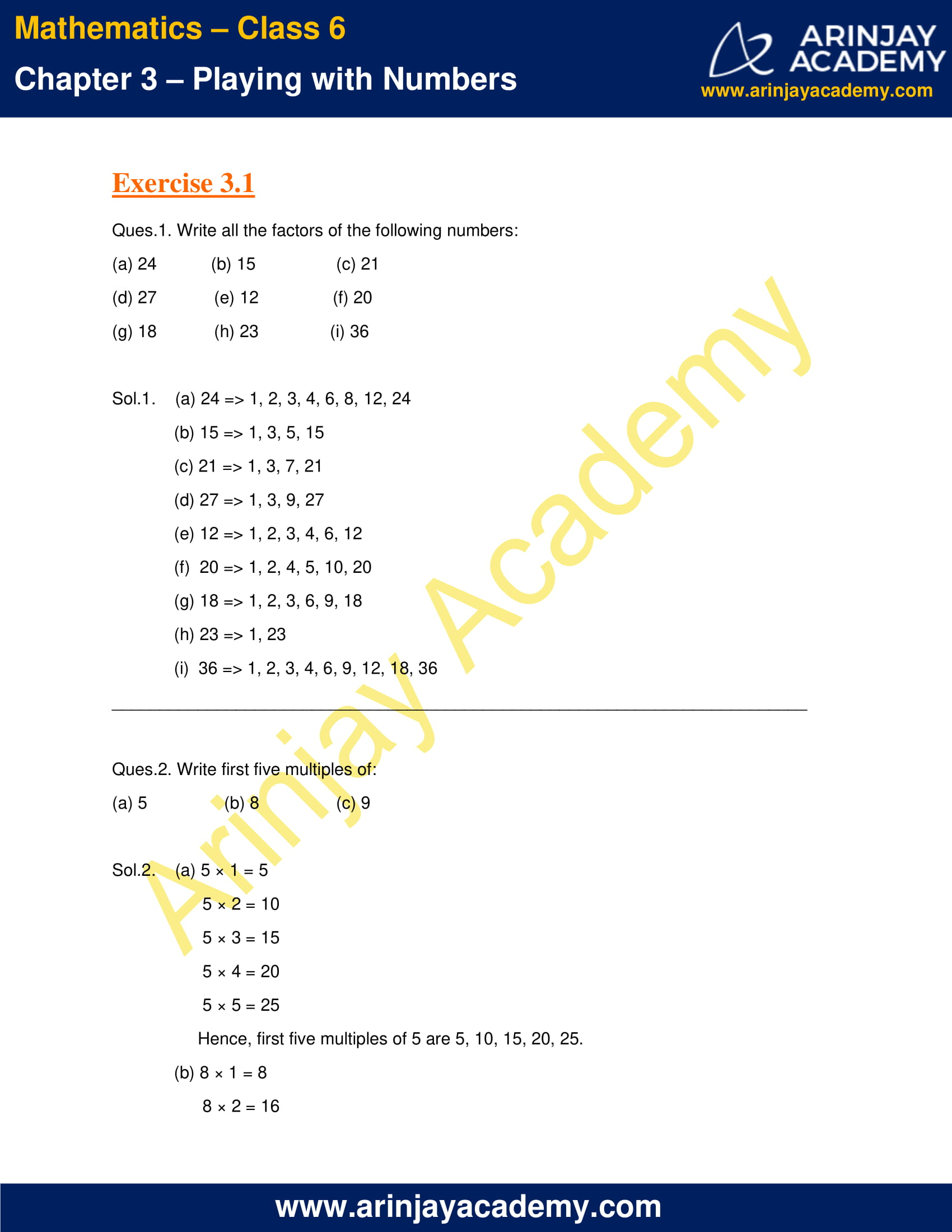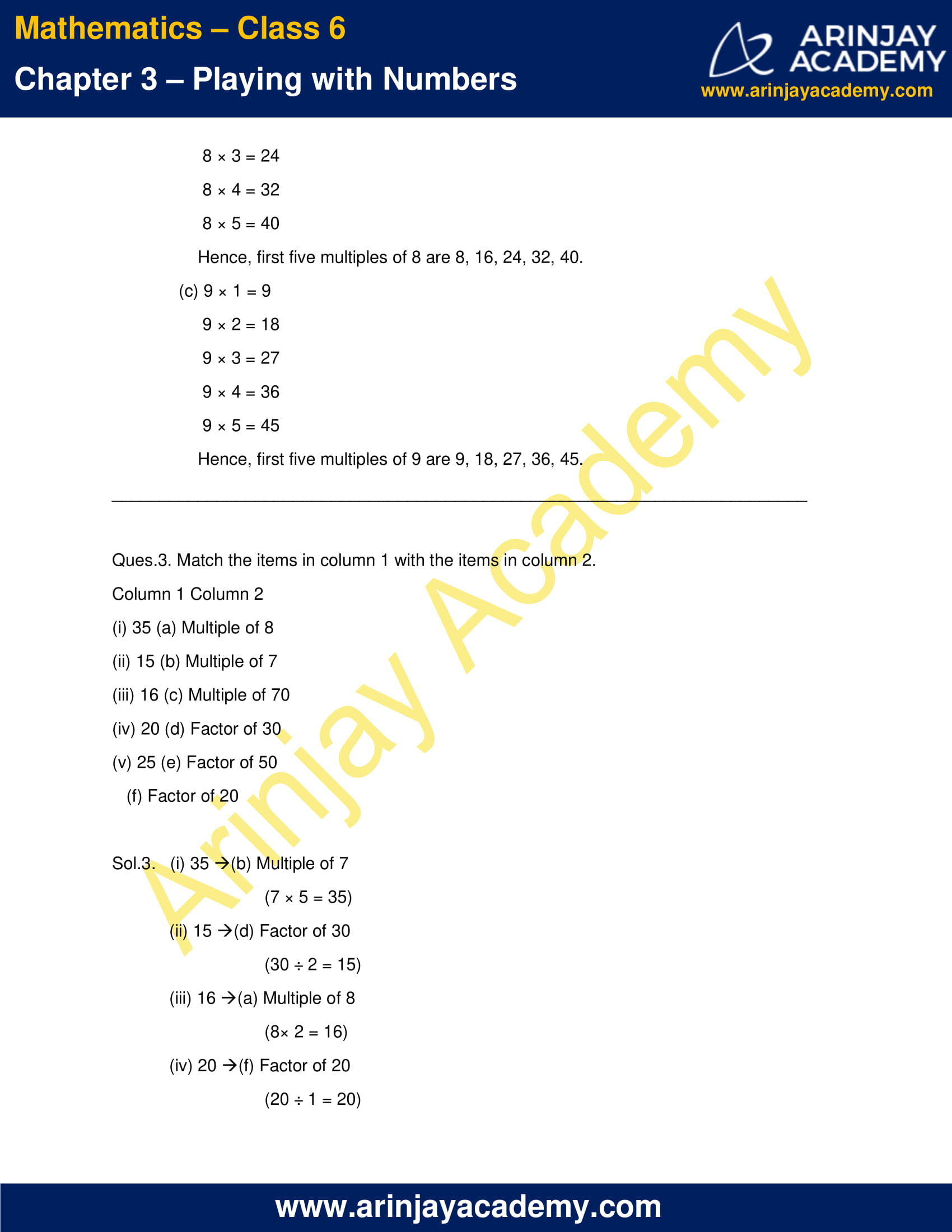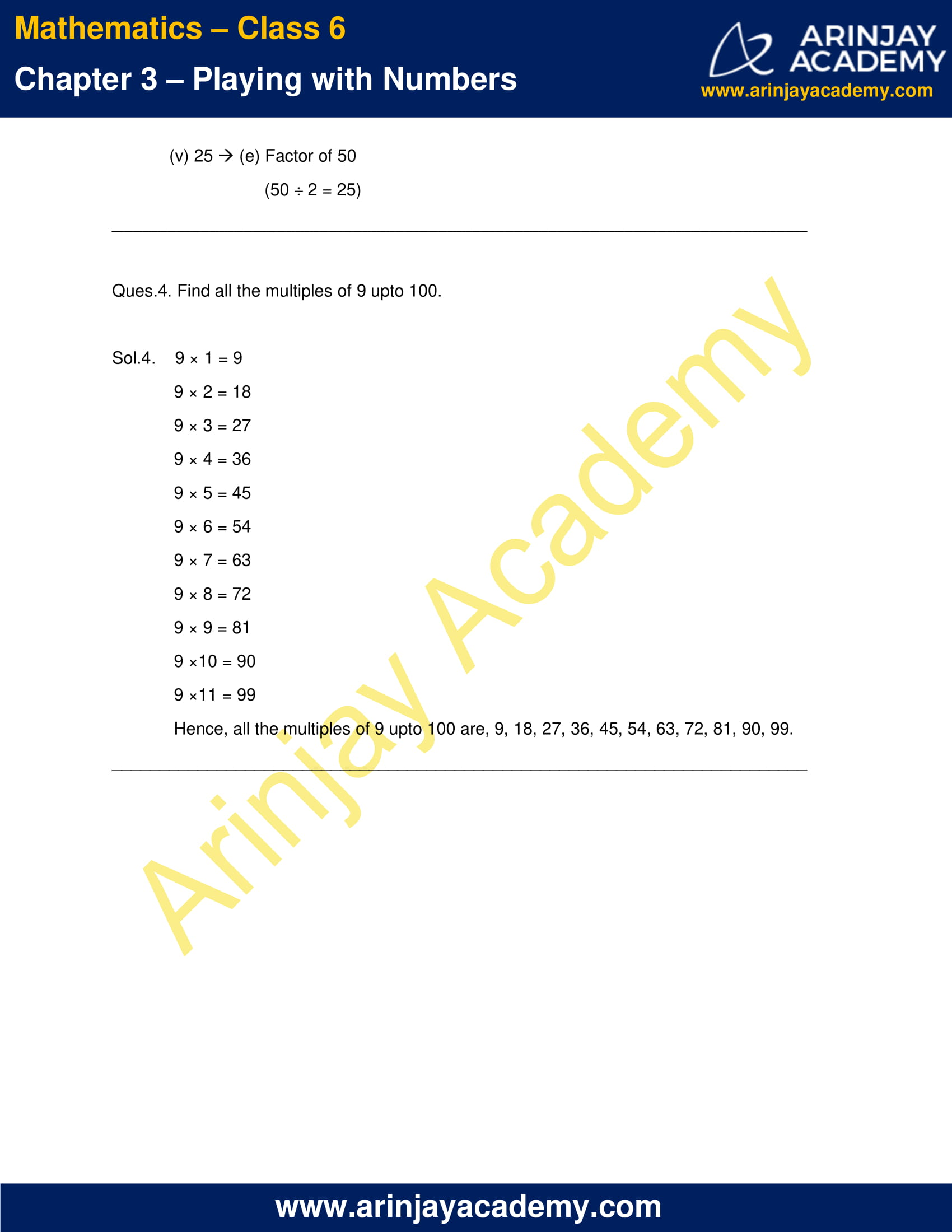### NCERT Solutions for Class 6 Maths Chapter 3 Exercise 3.2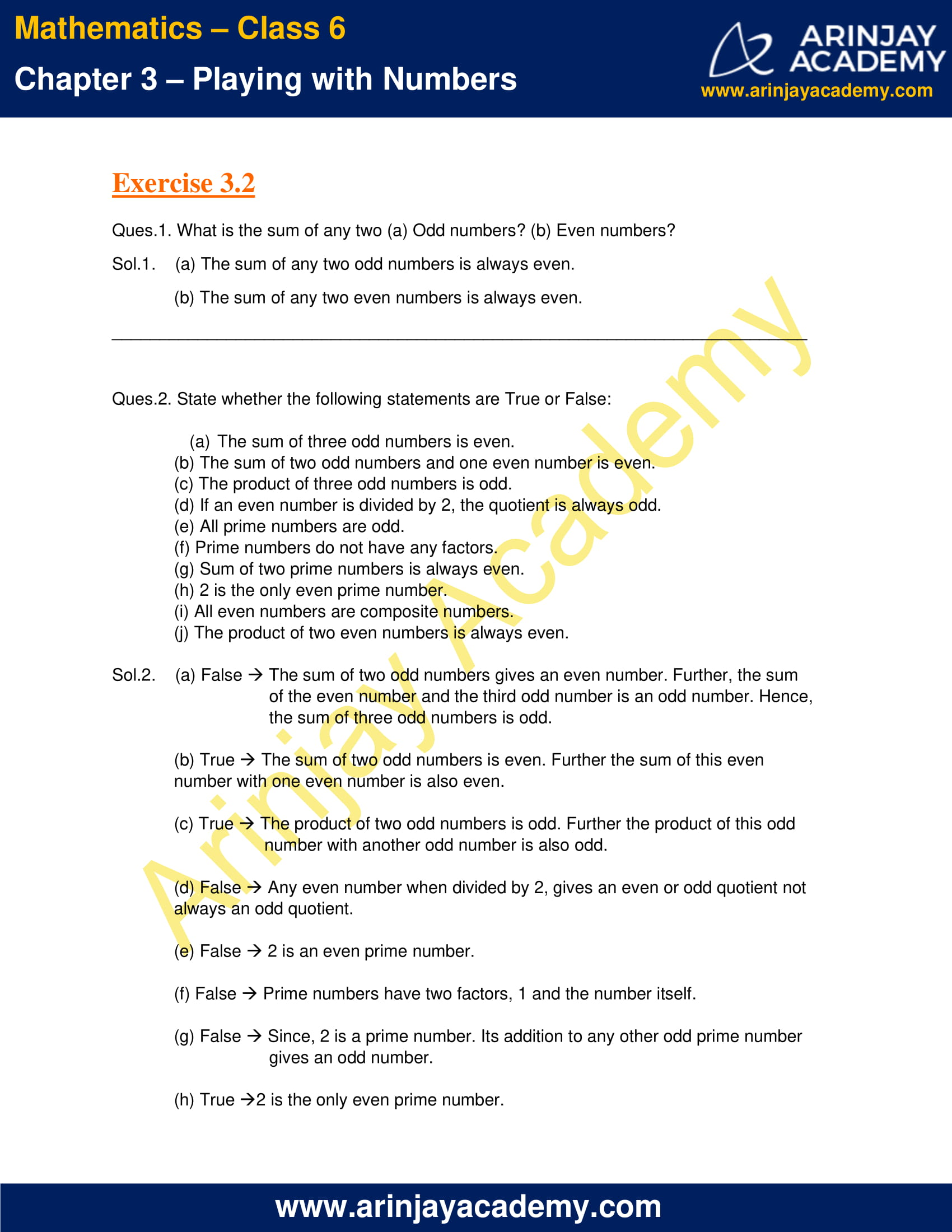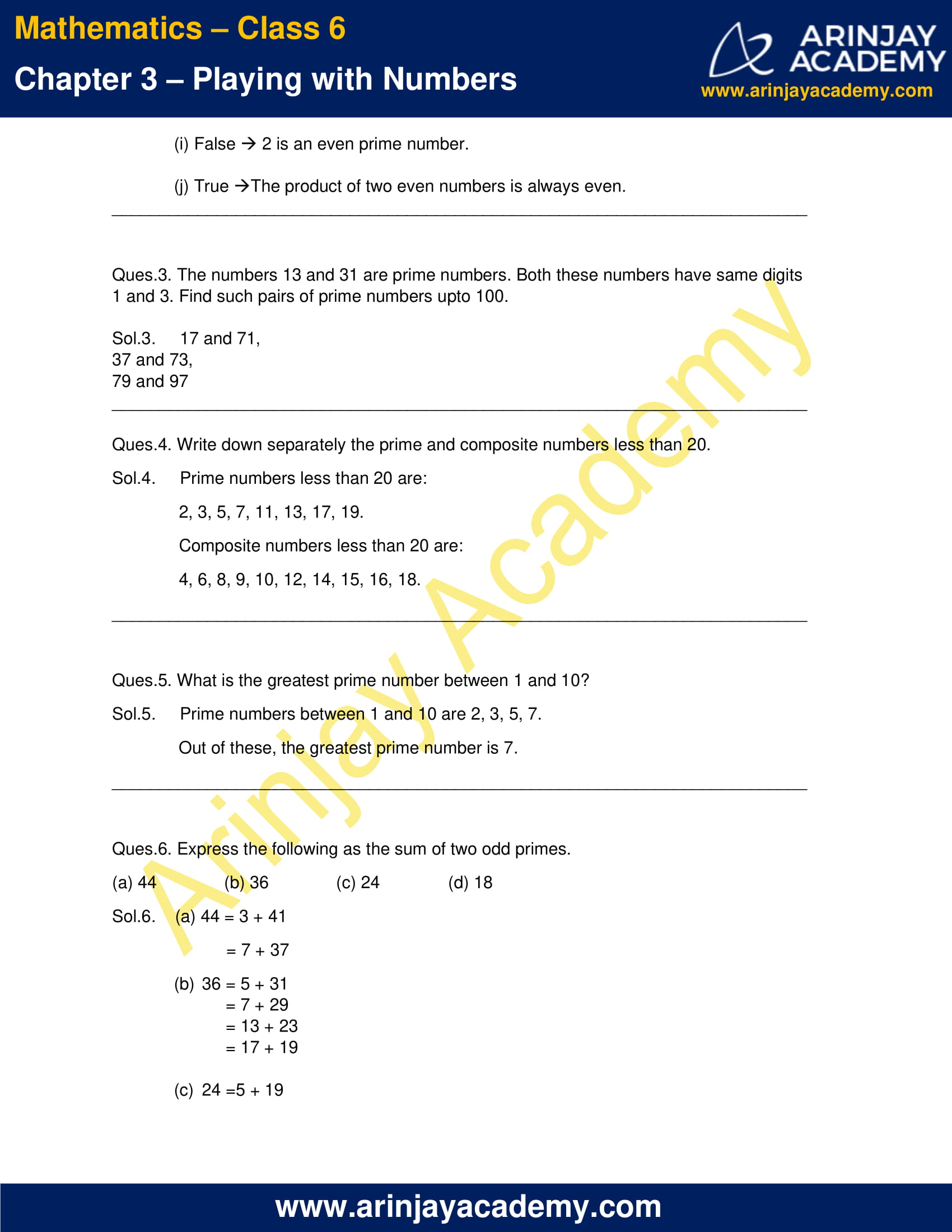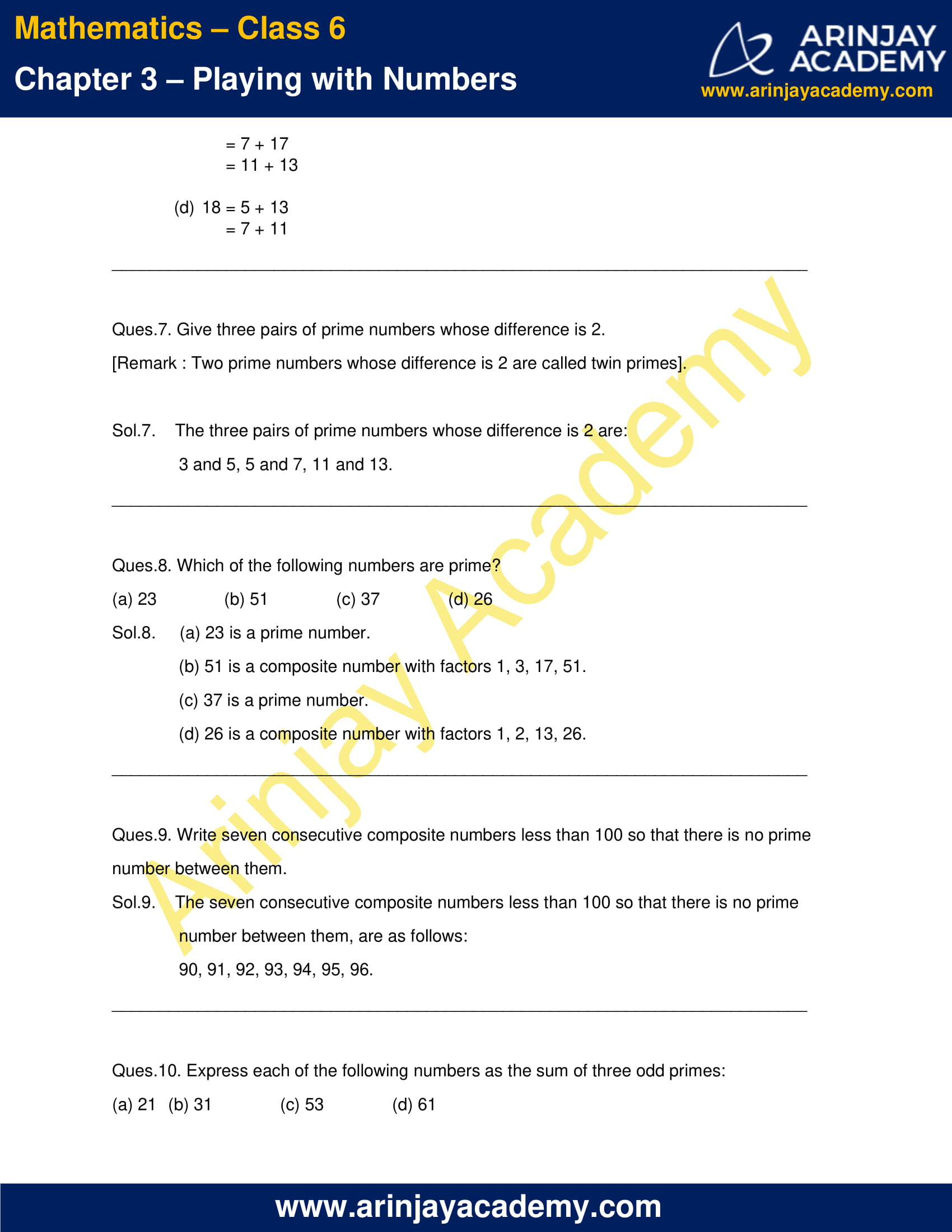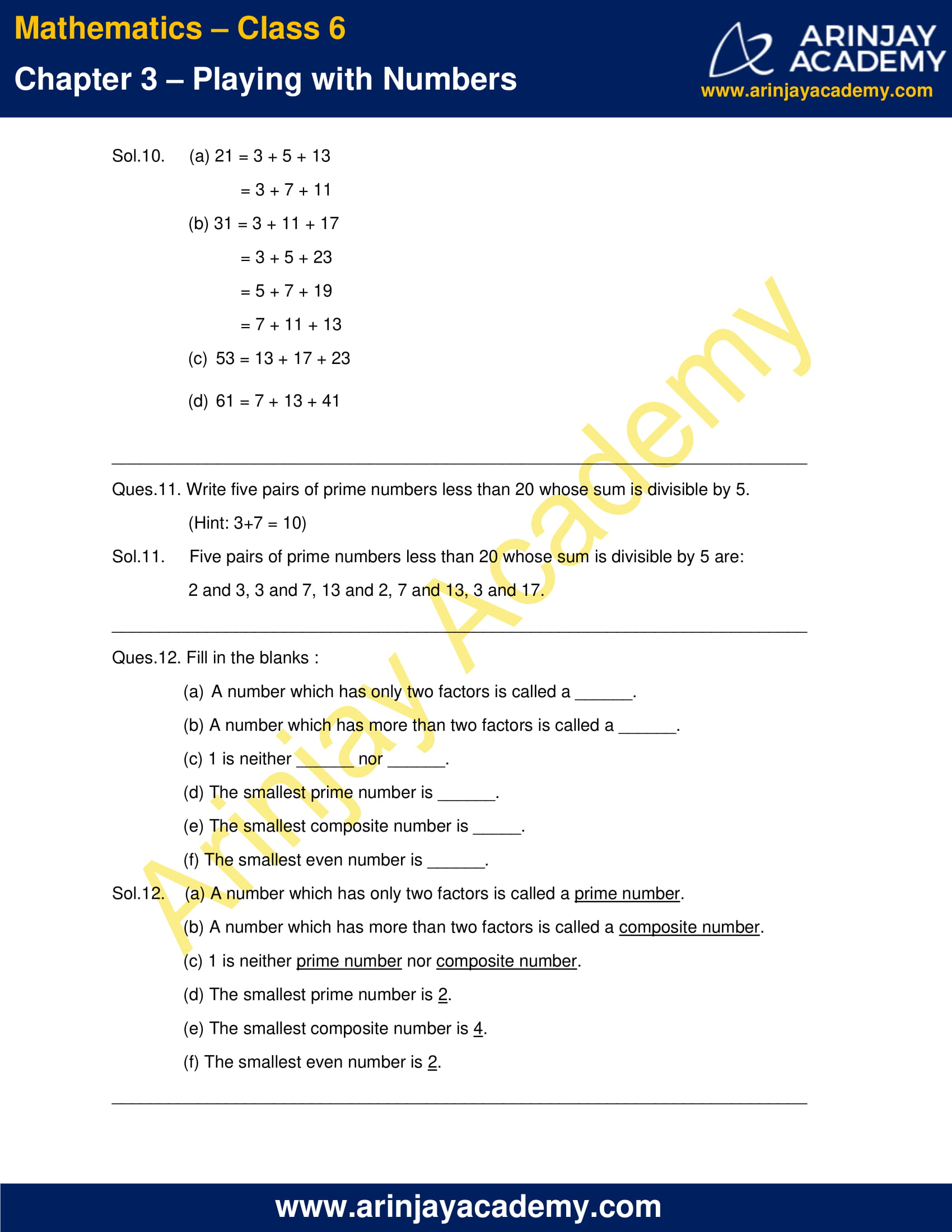### NCERT Solutions for Class 6 Maths Chapter 3 Exercise 3.3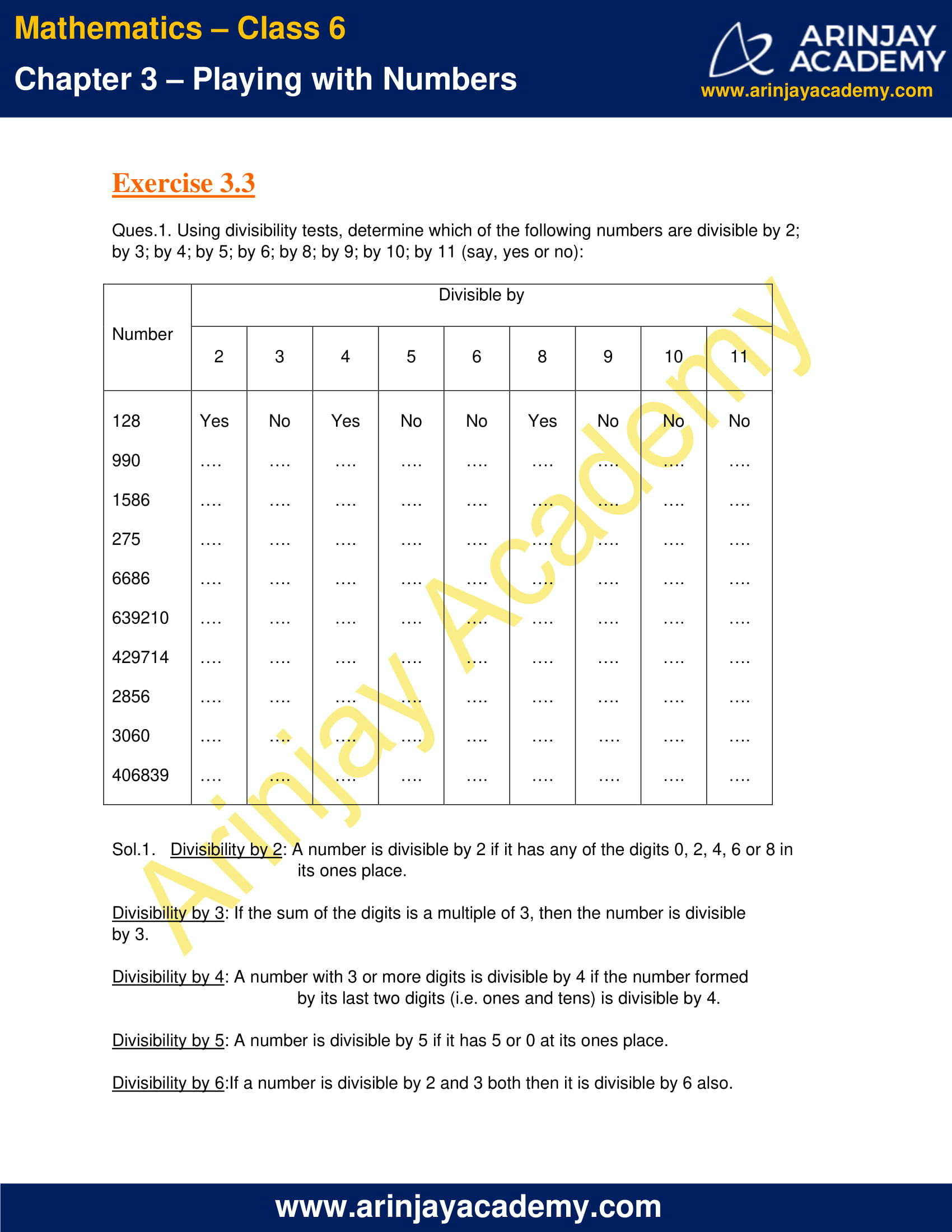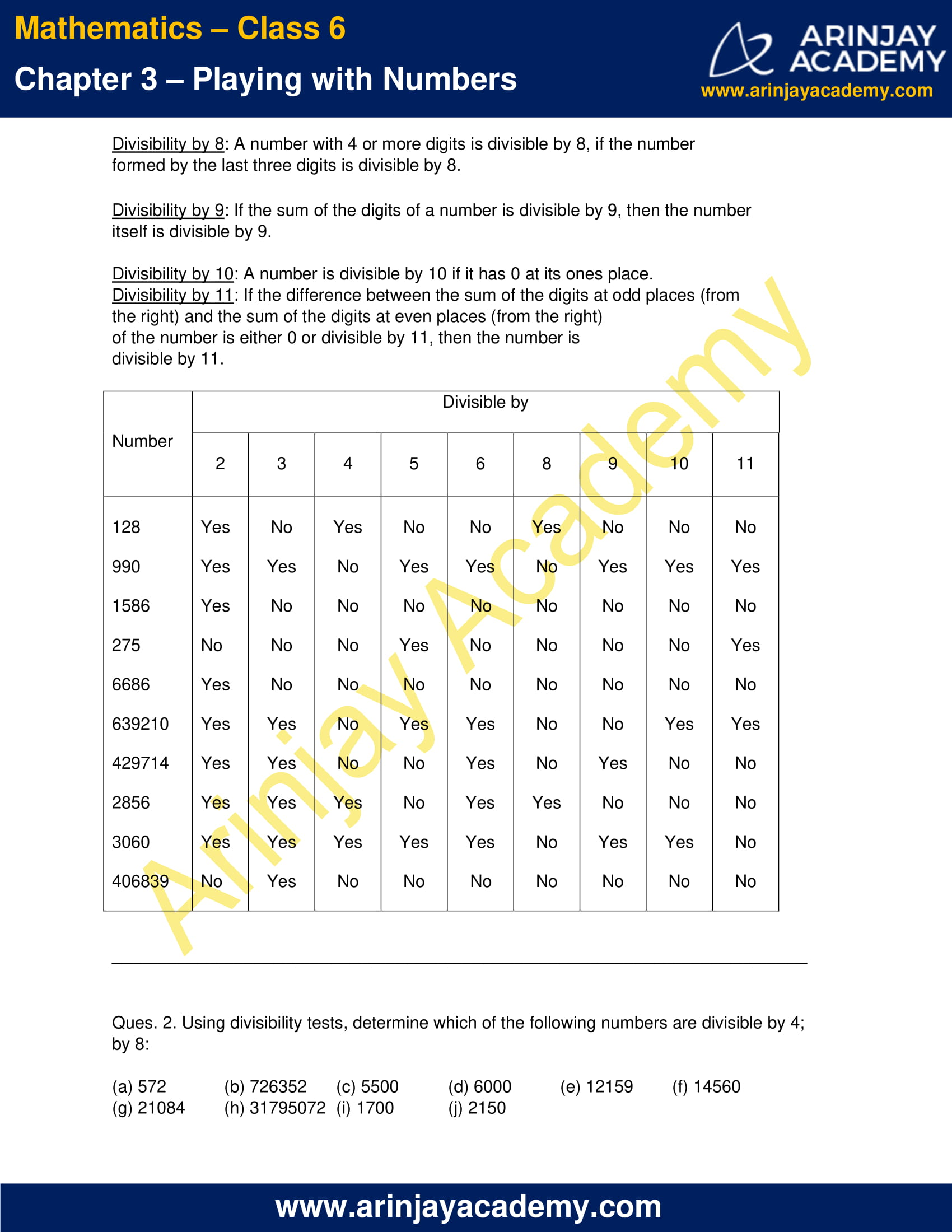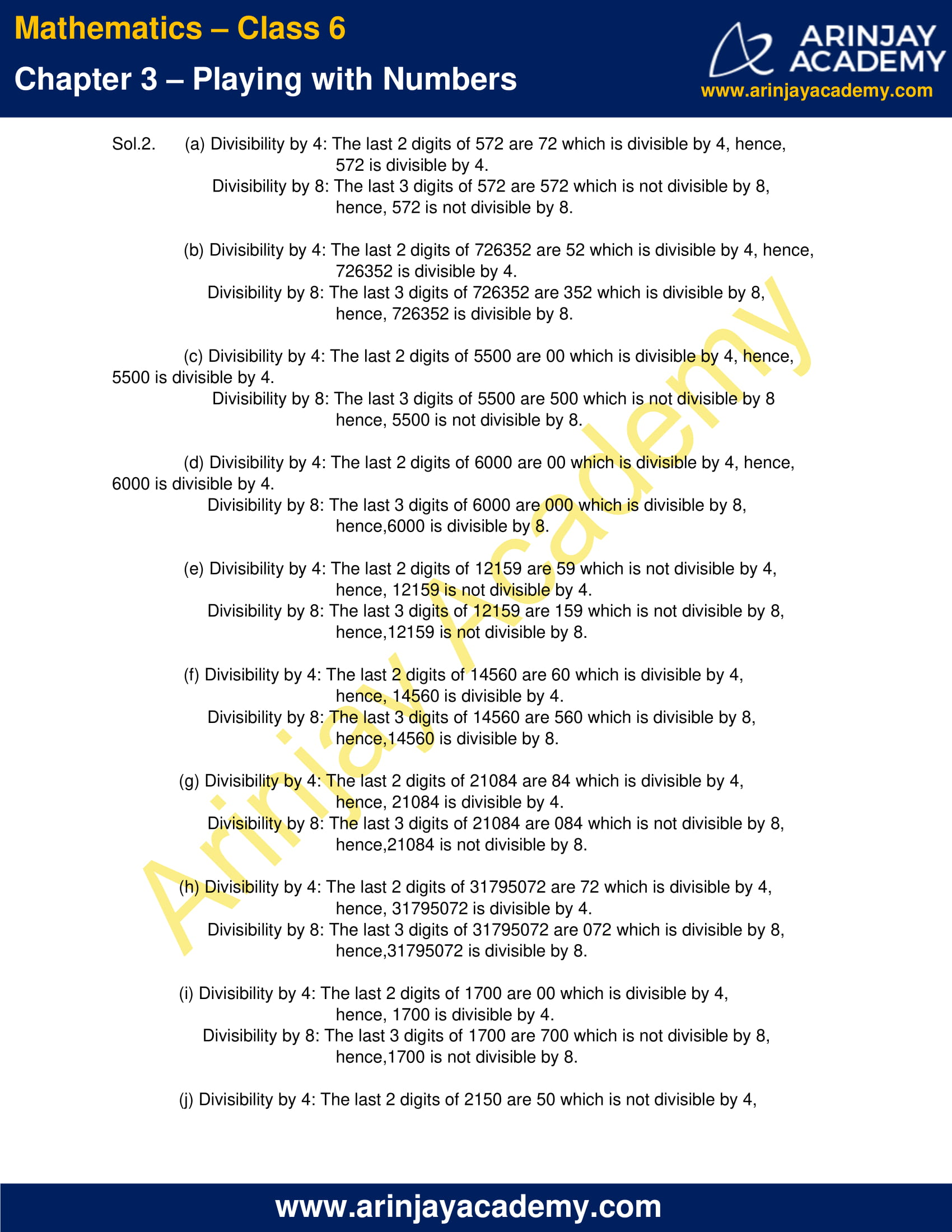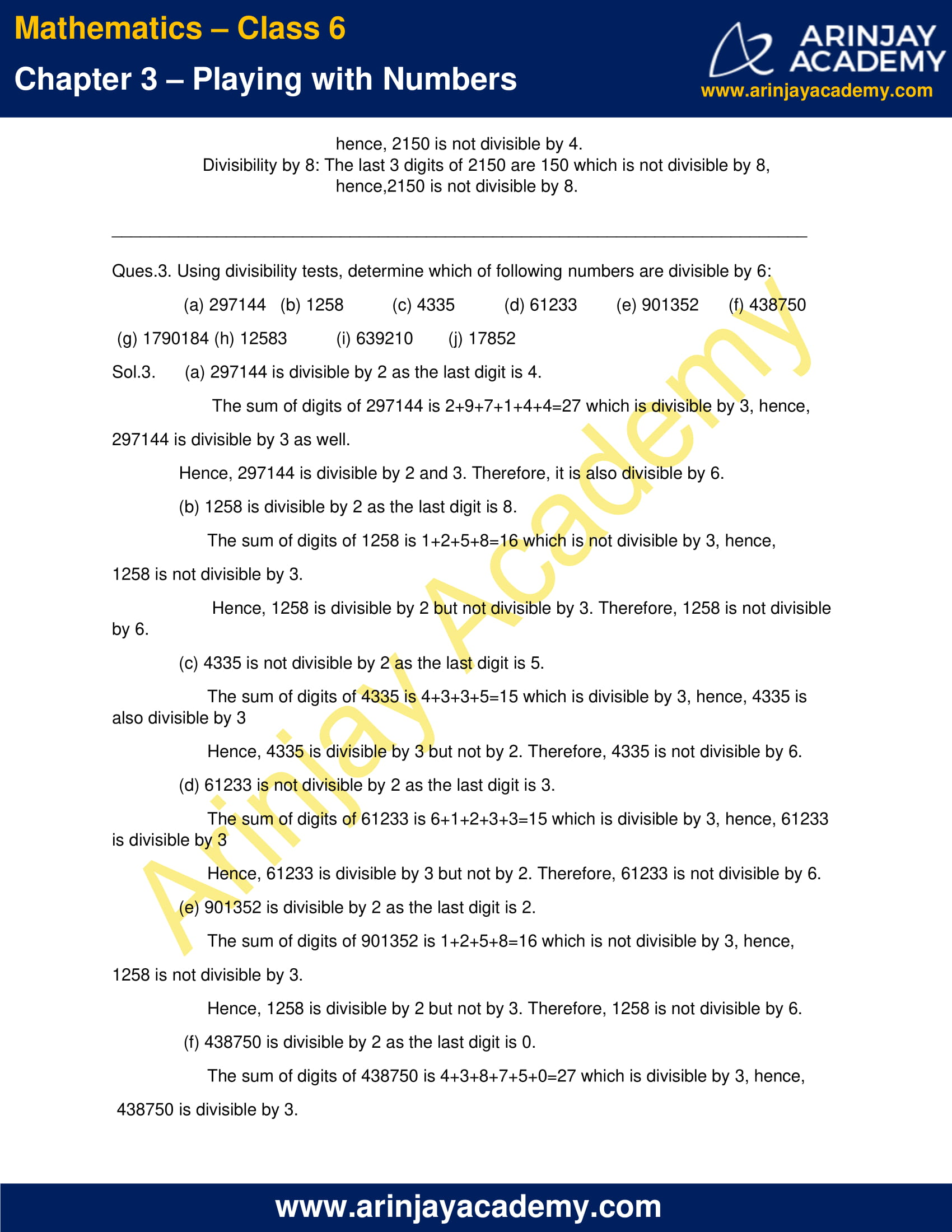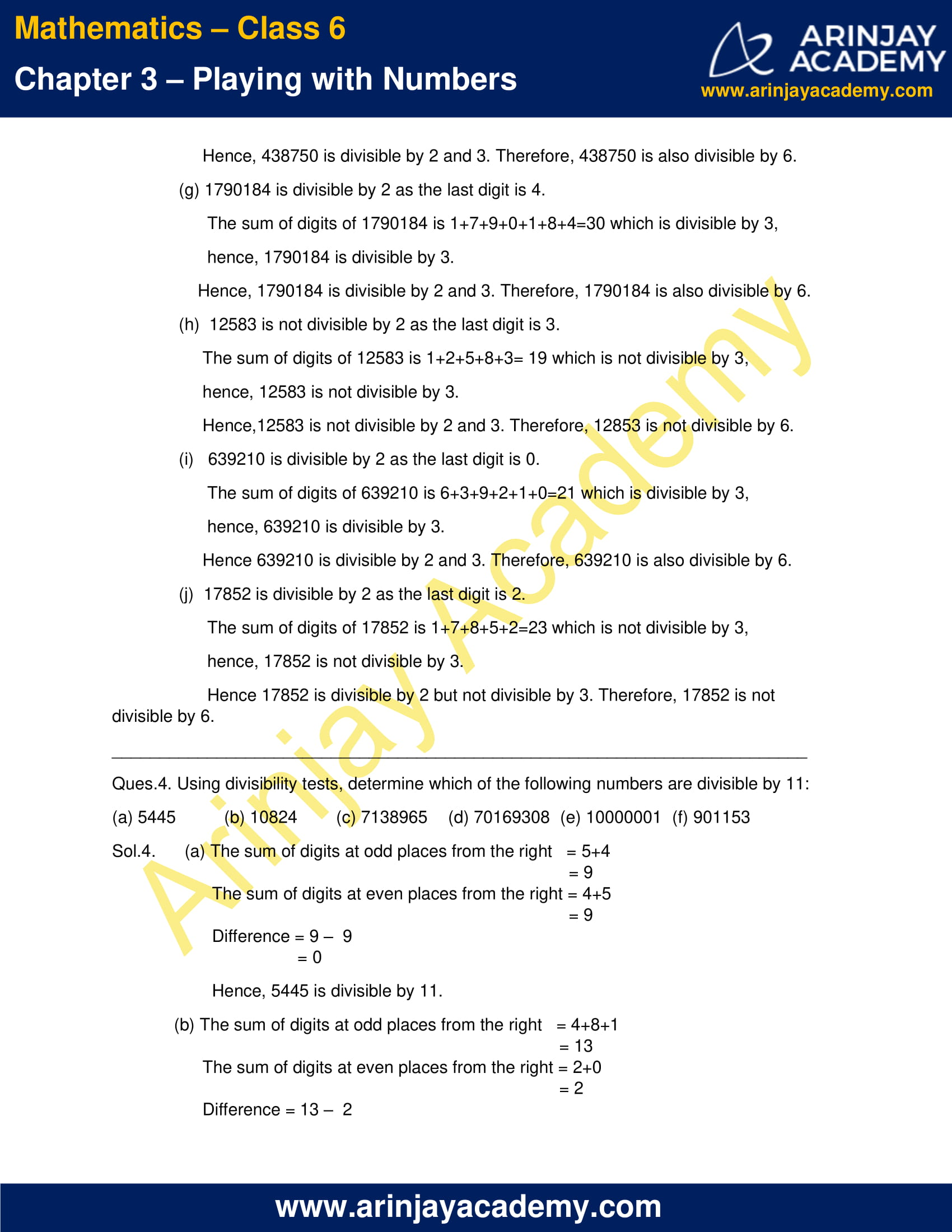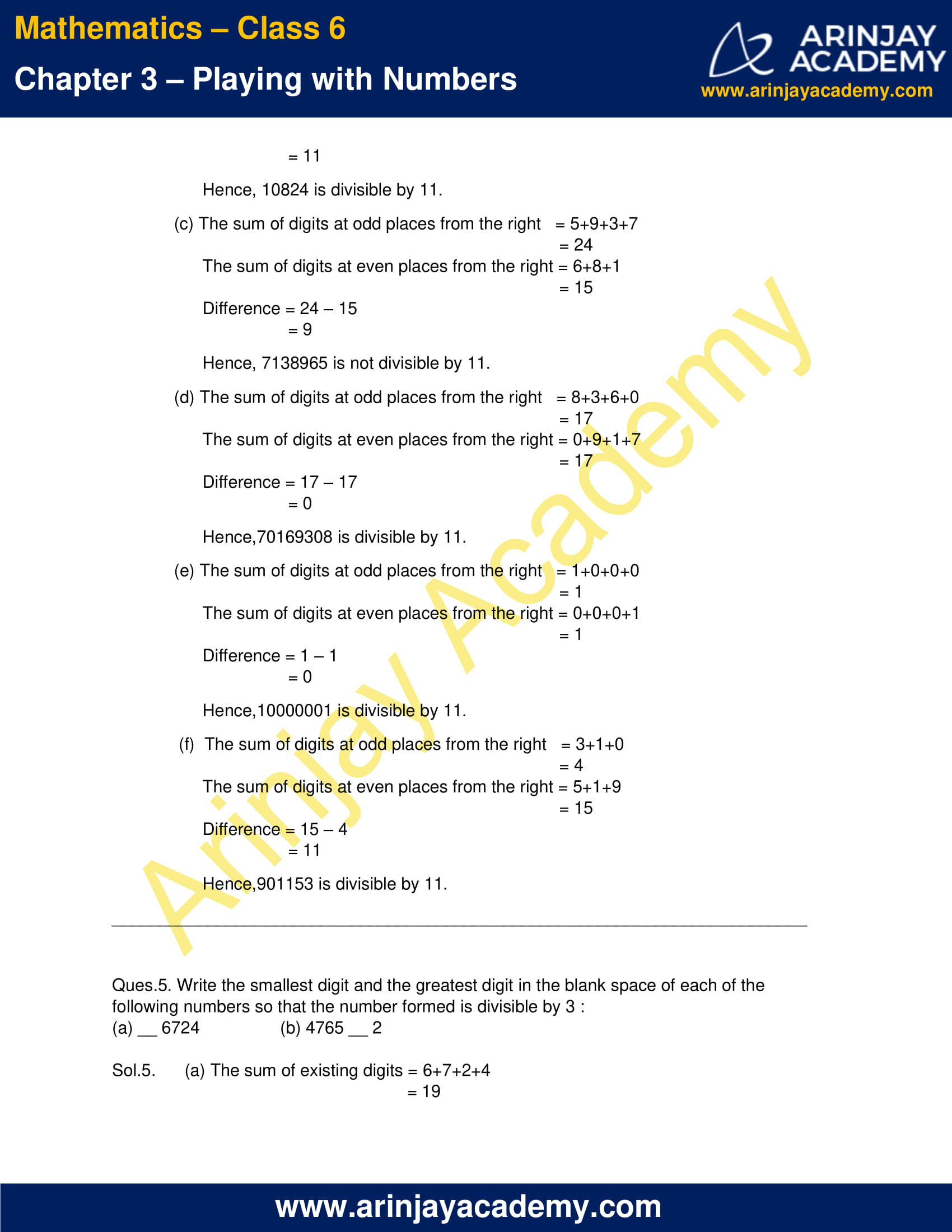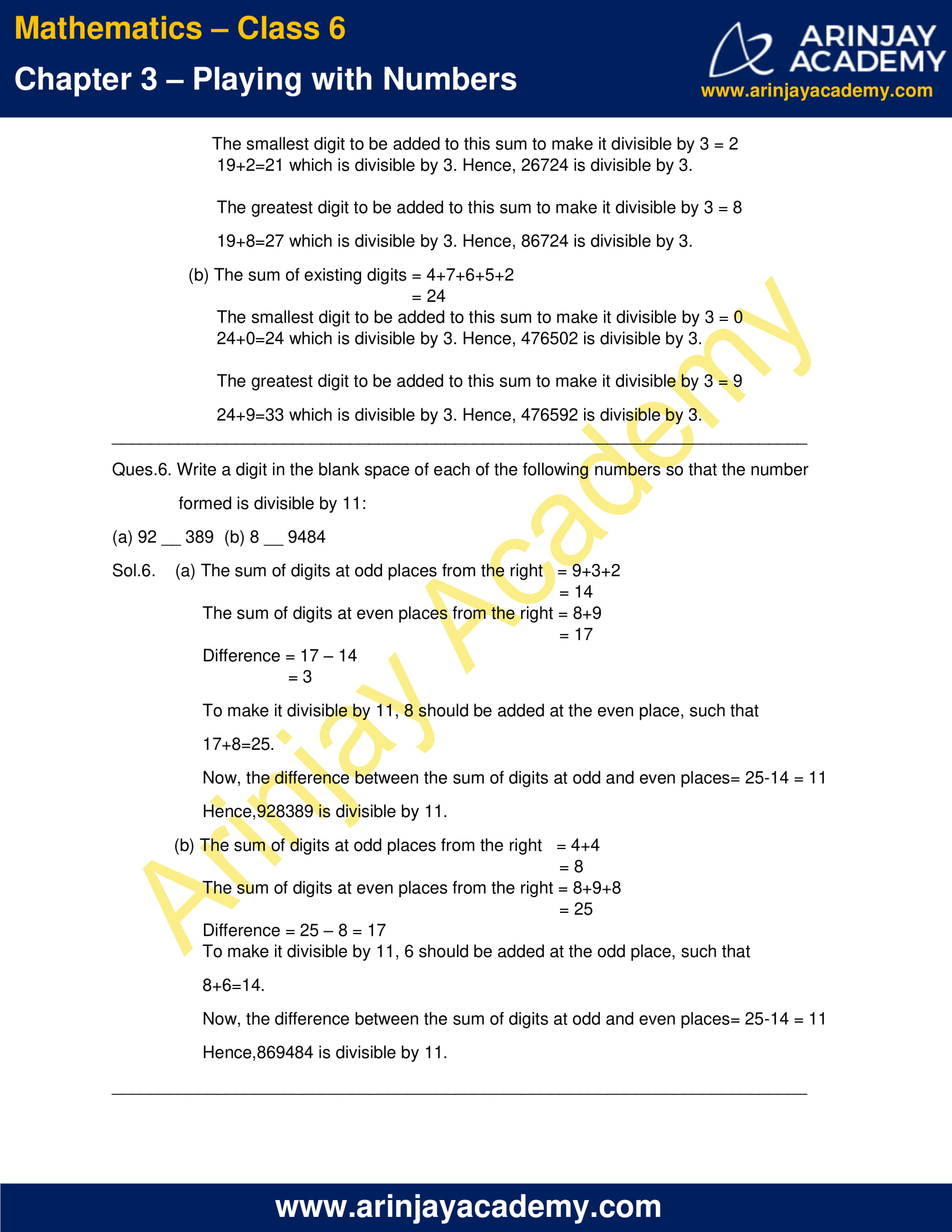### NCERT Solutions for Class 6 Maths Chapter 3 Exercise 3.4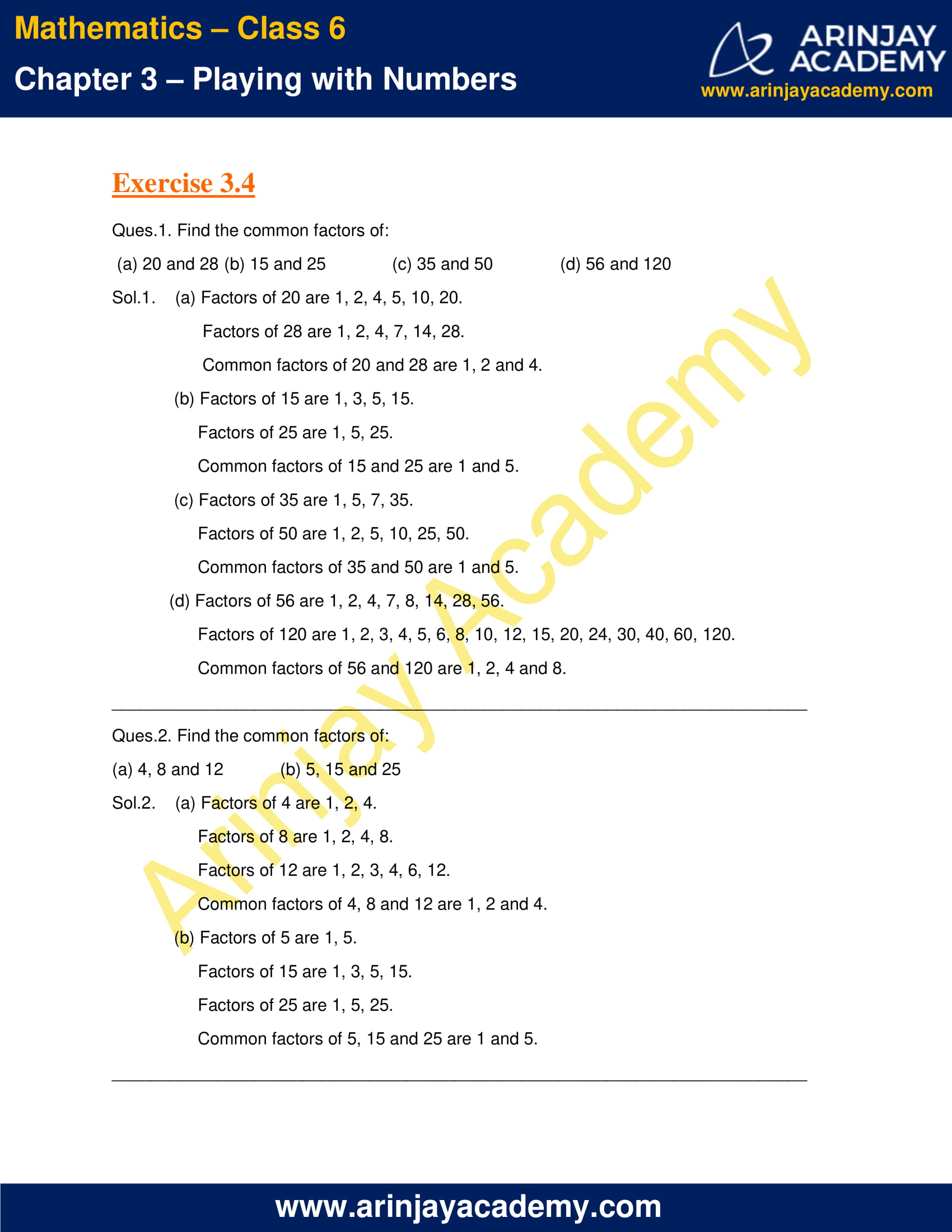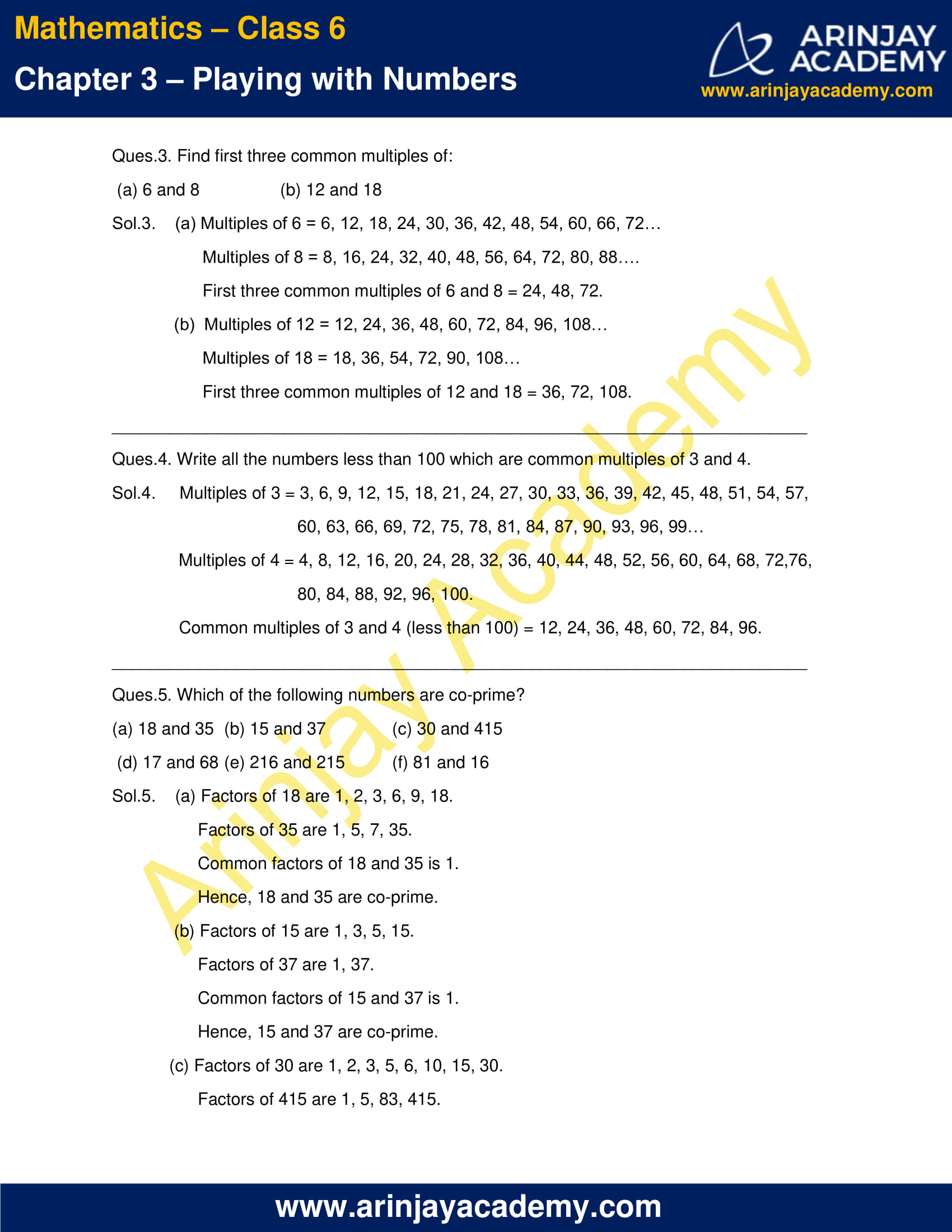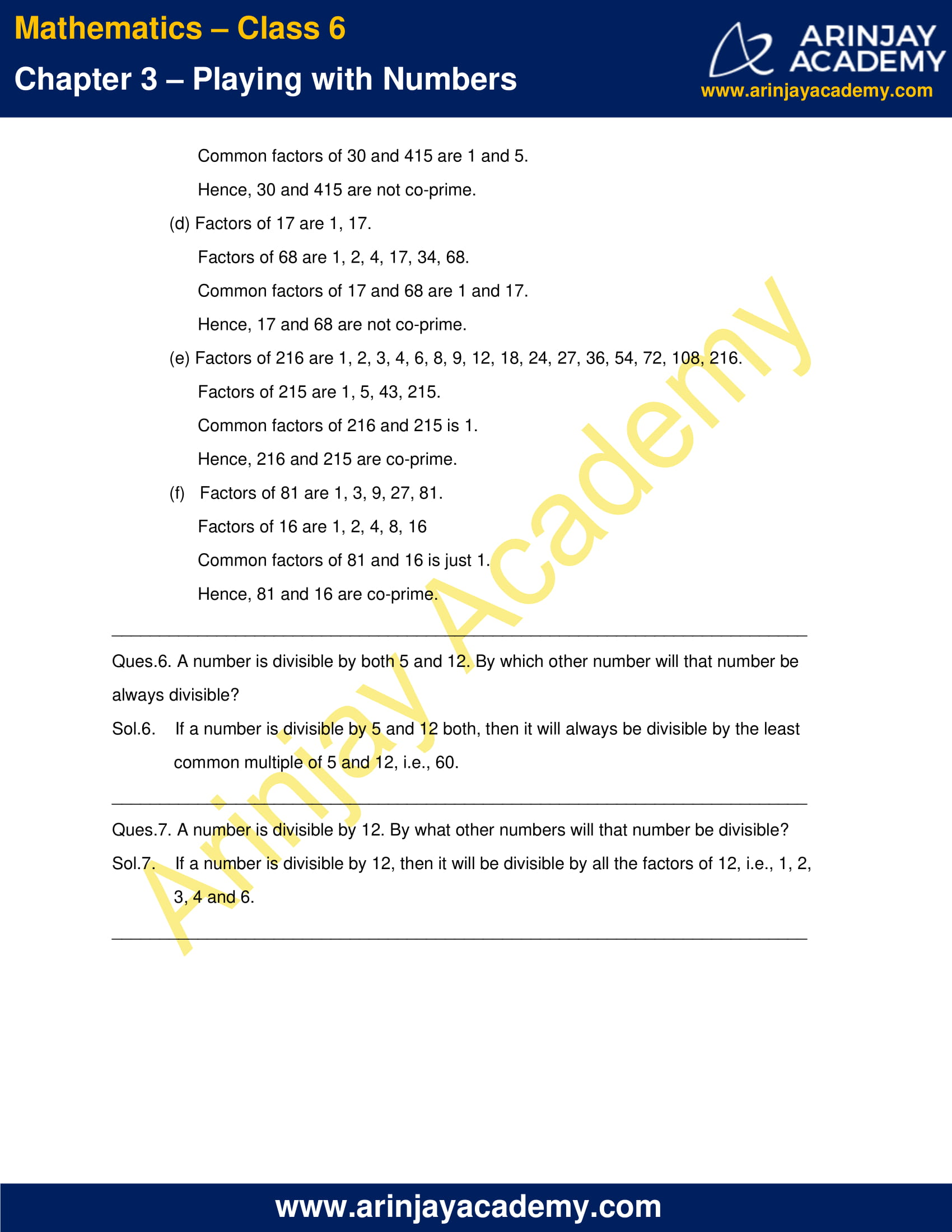### NCERT Solutions for Class 6 Maths Chapter 3 Exercise 3.5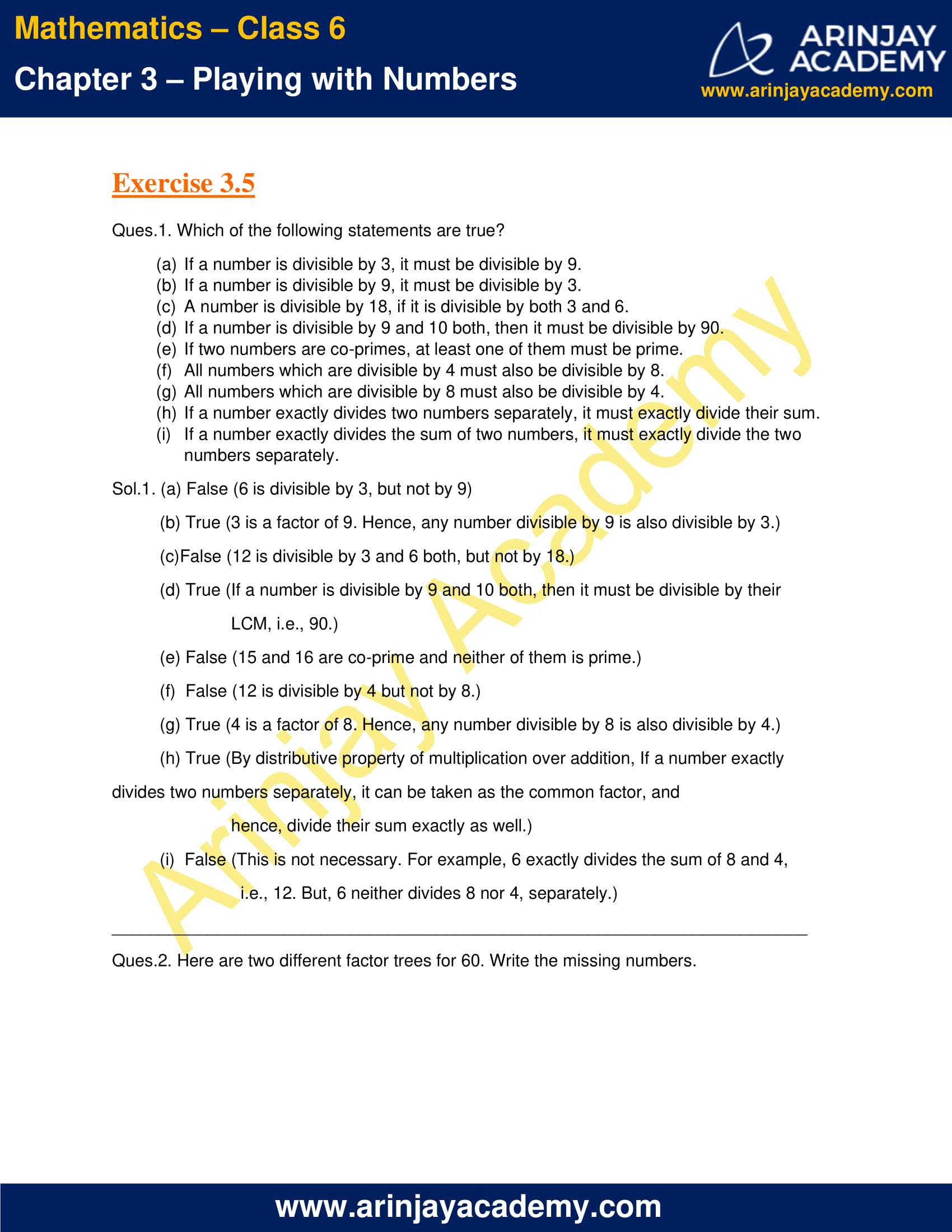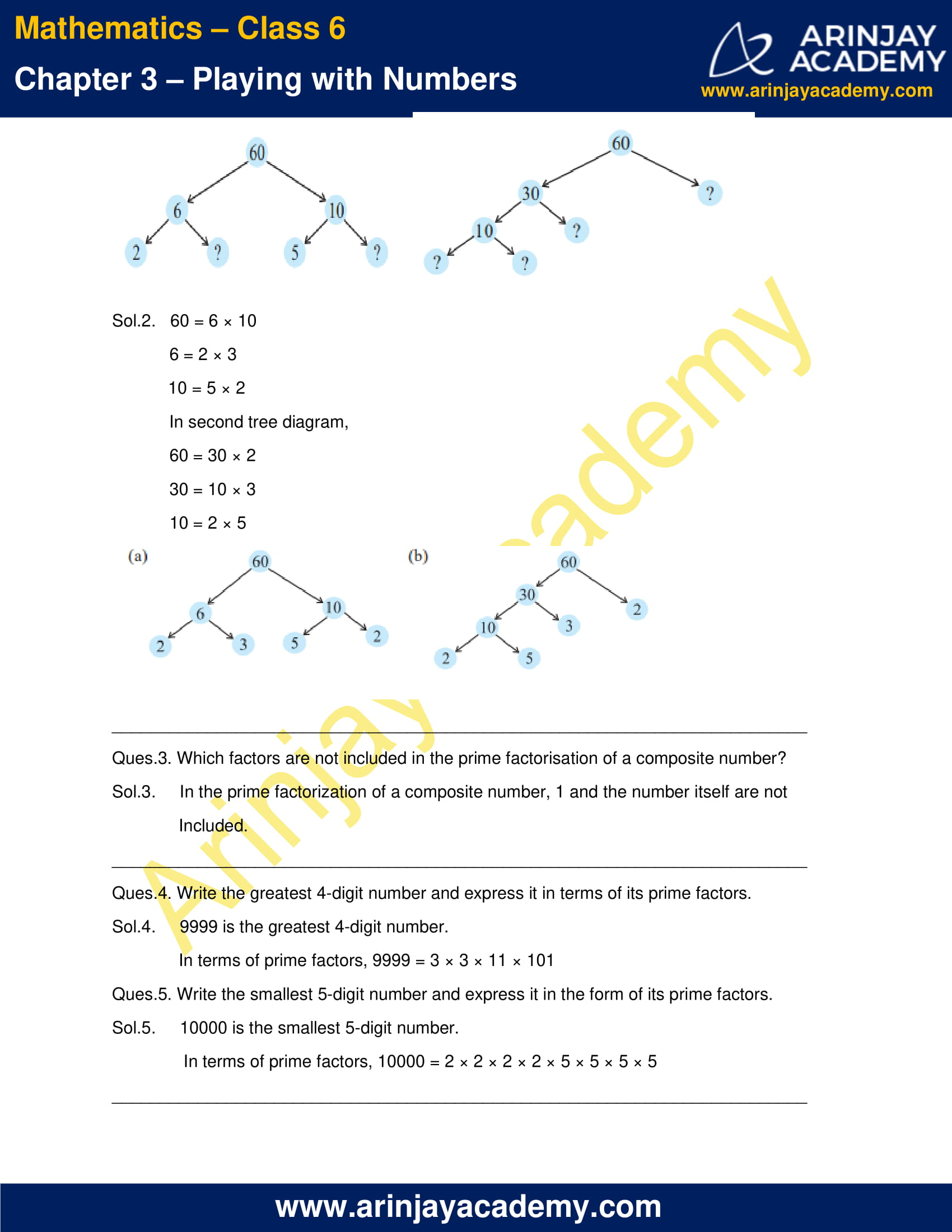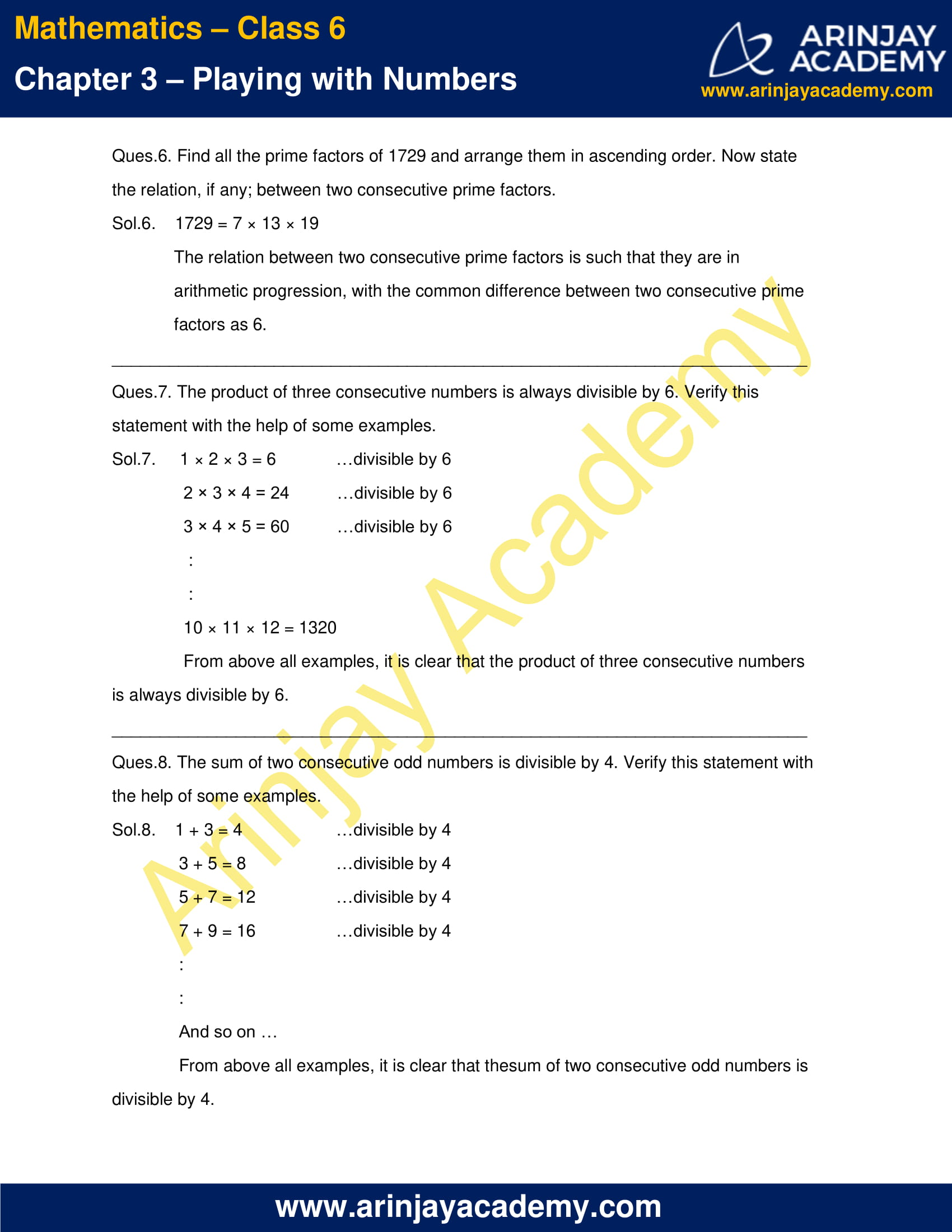### NCERT Solutions for Class 6 Maths Chapter 3 Exercise 3.6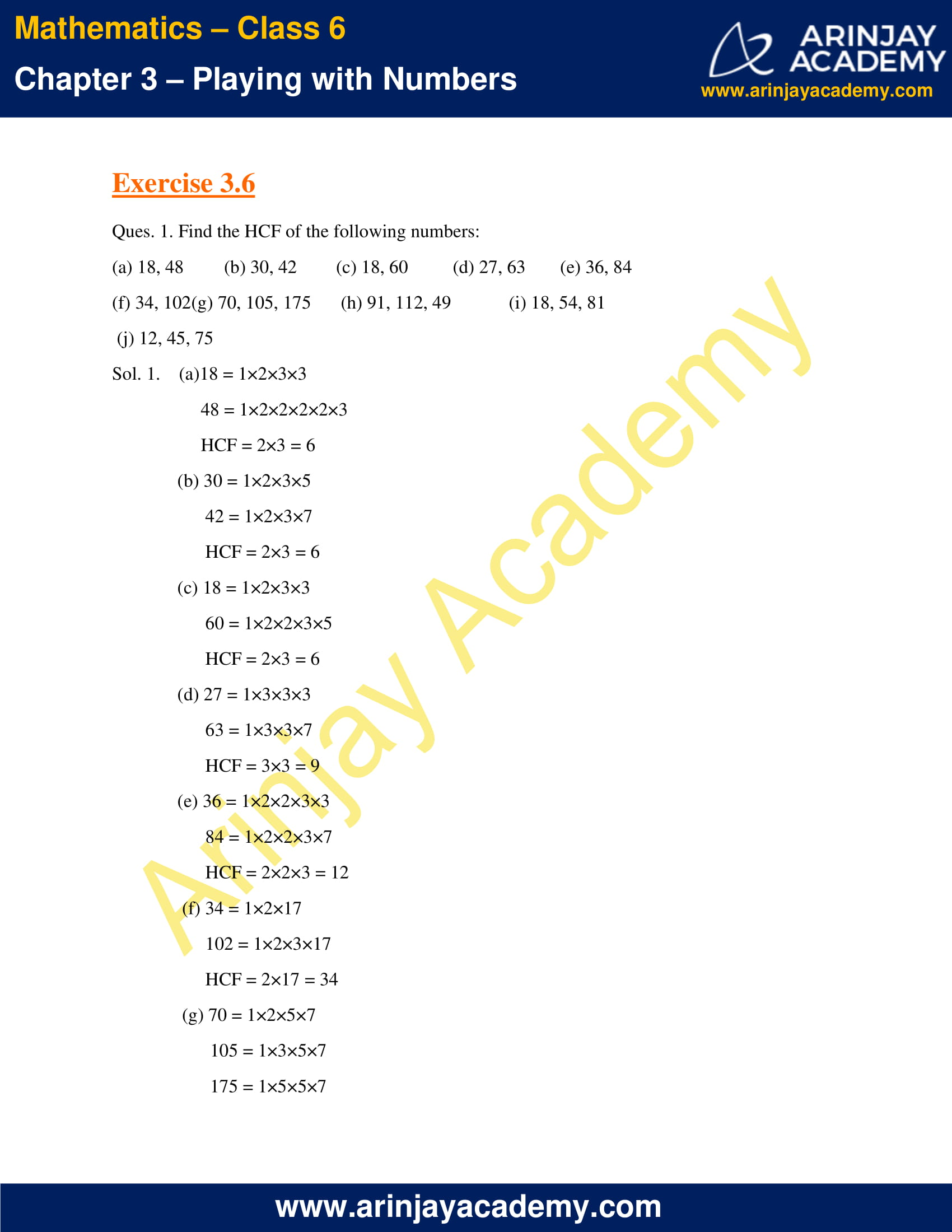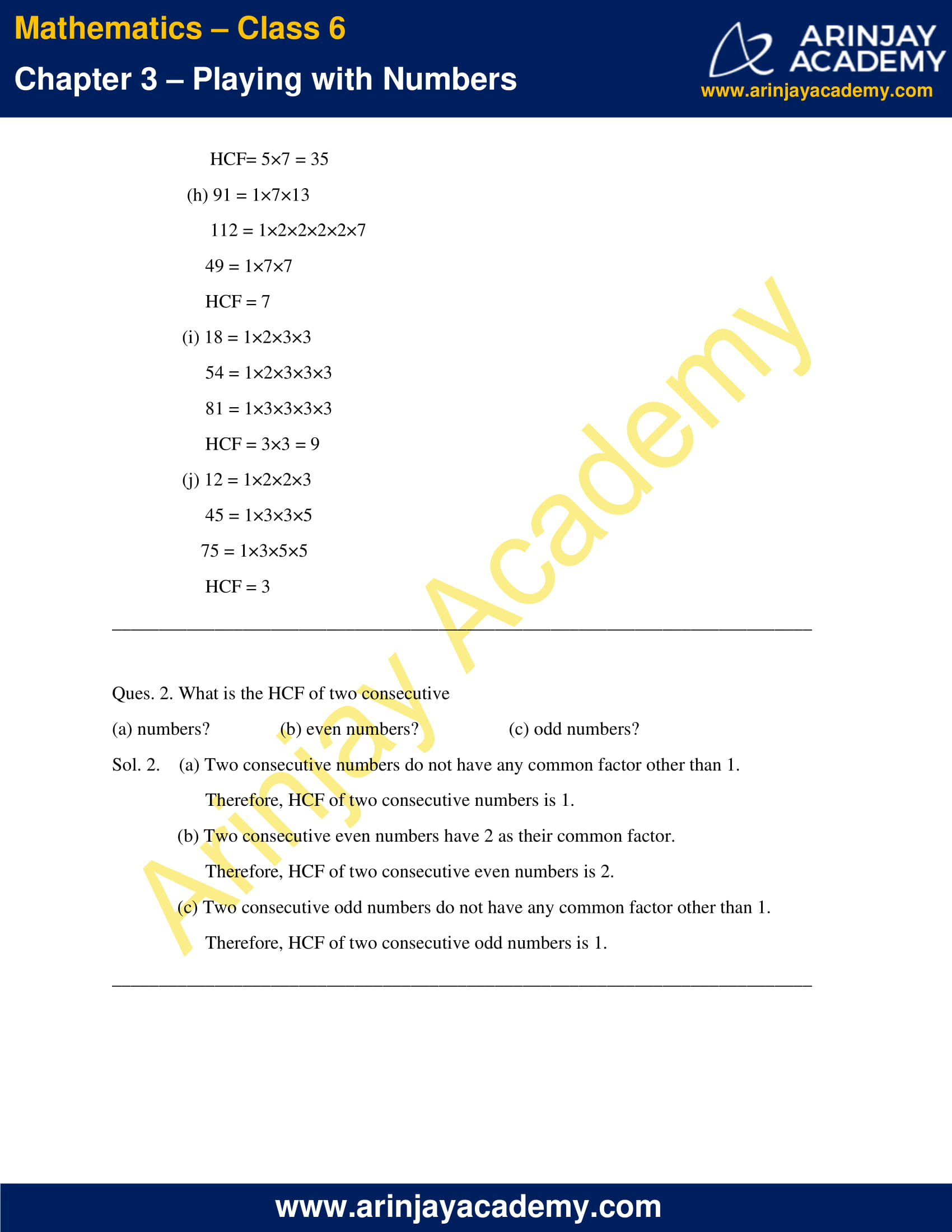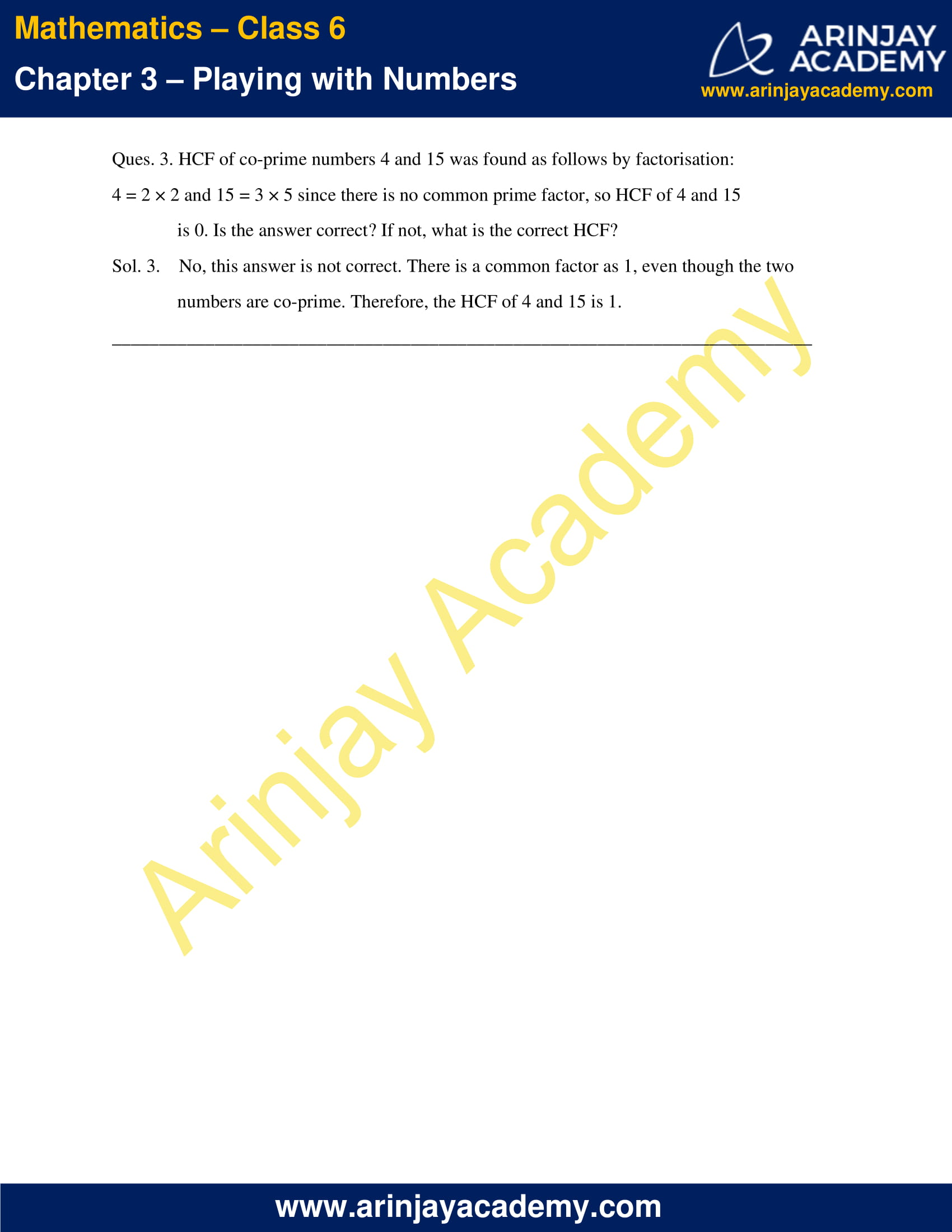### NCERT Solutions for Class 6 Maths Chapter 3 Exercise 3.7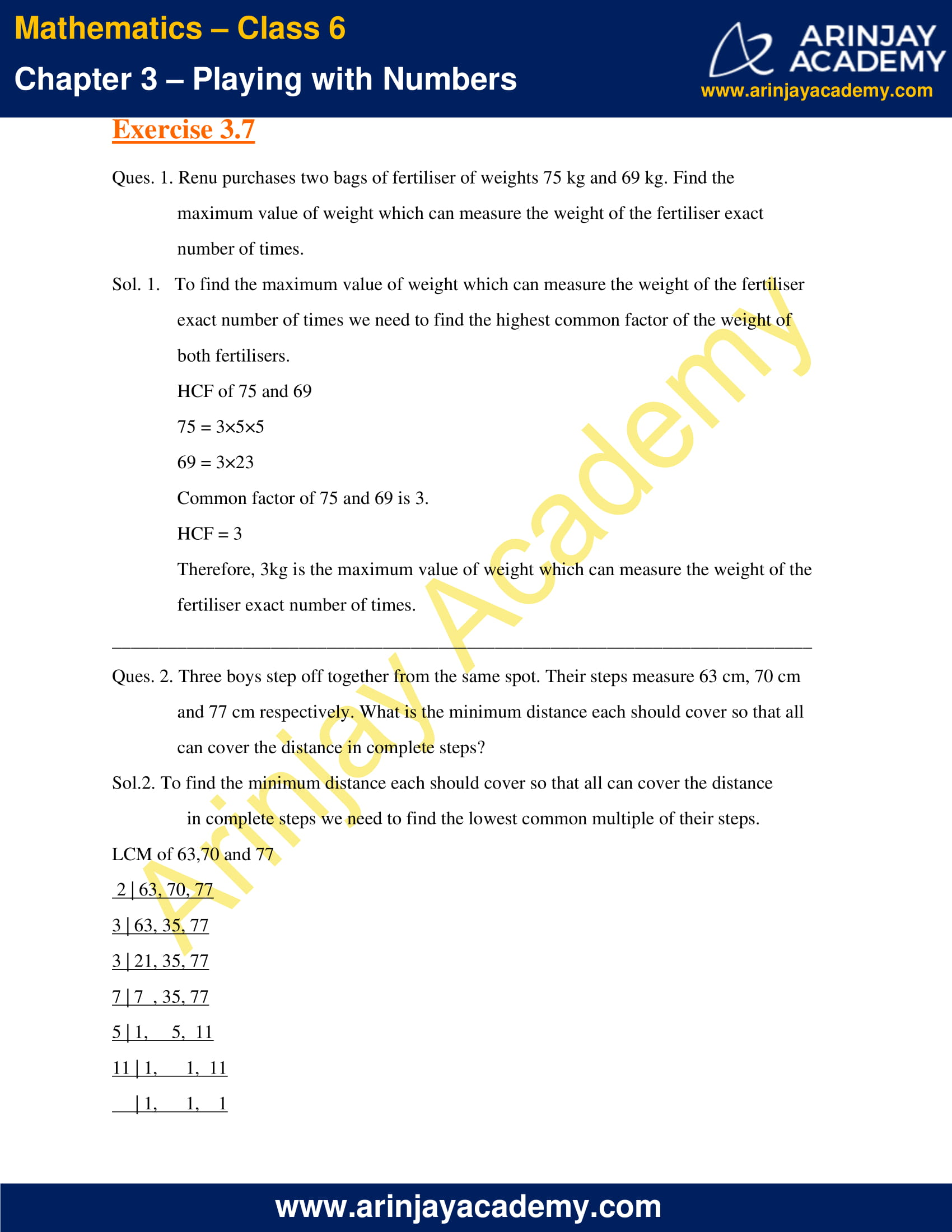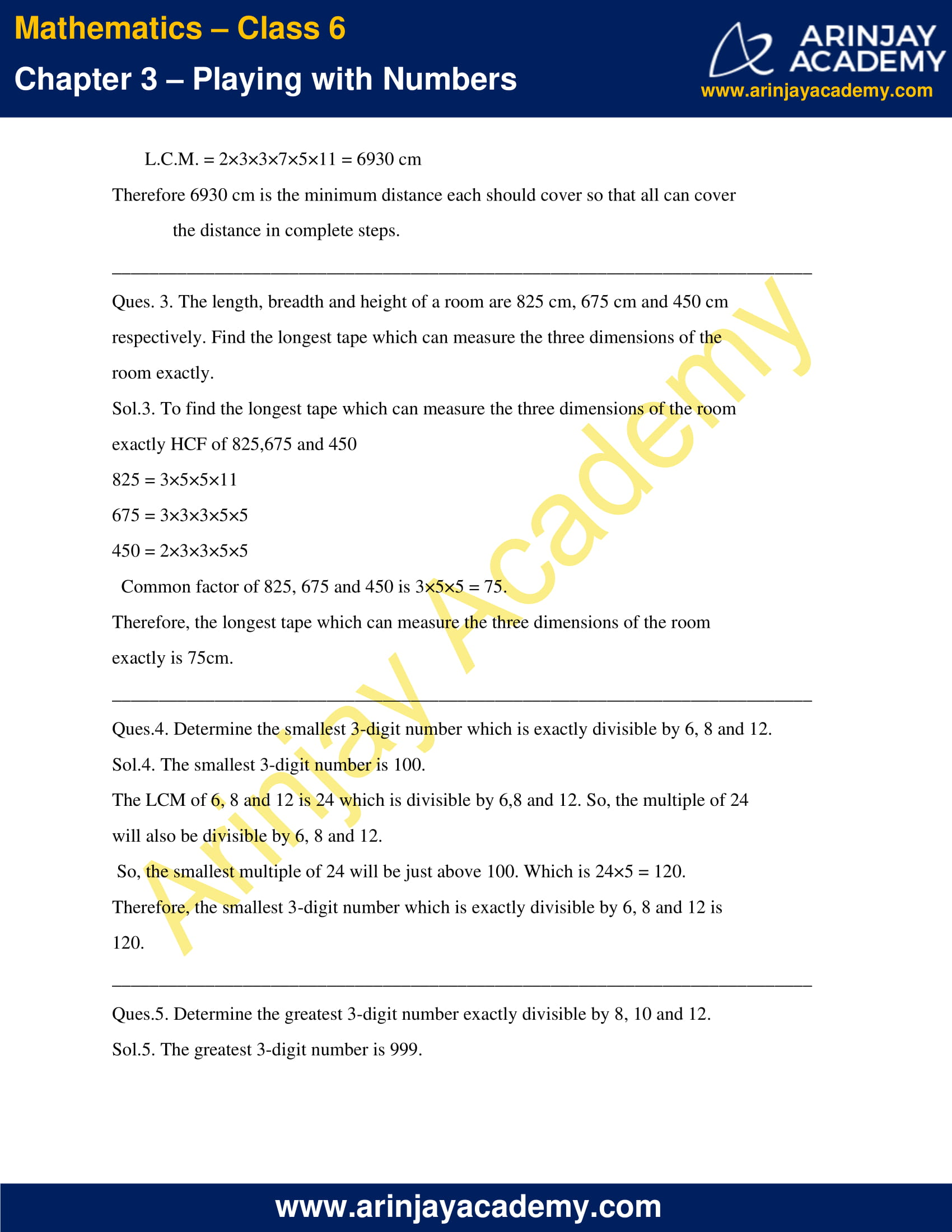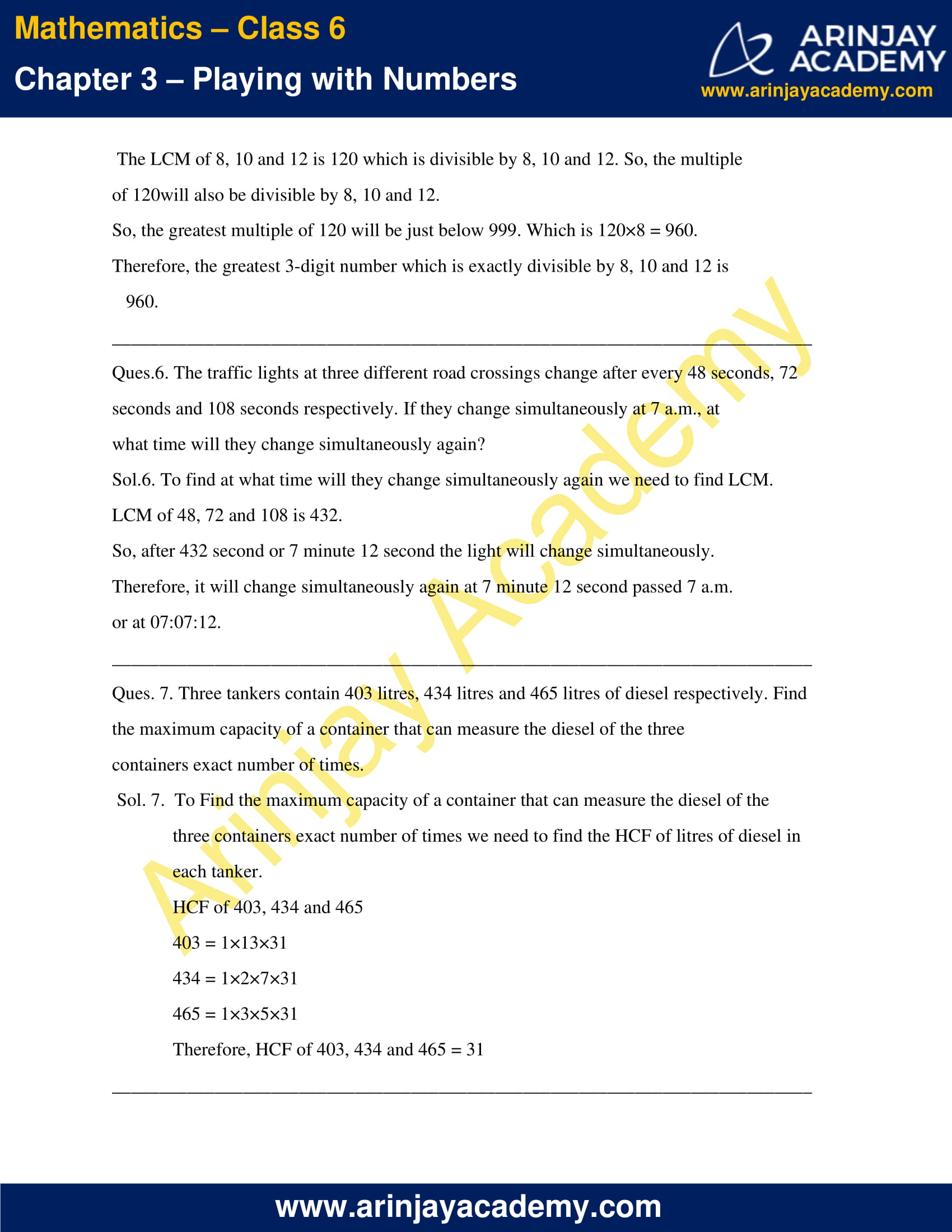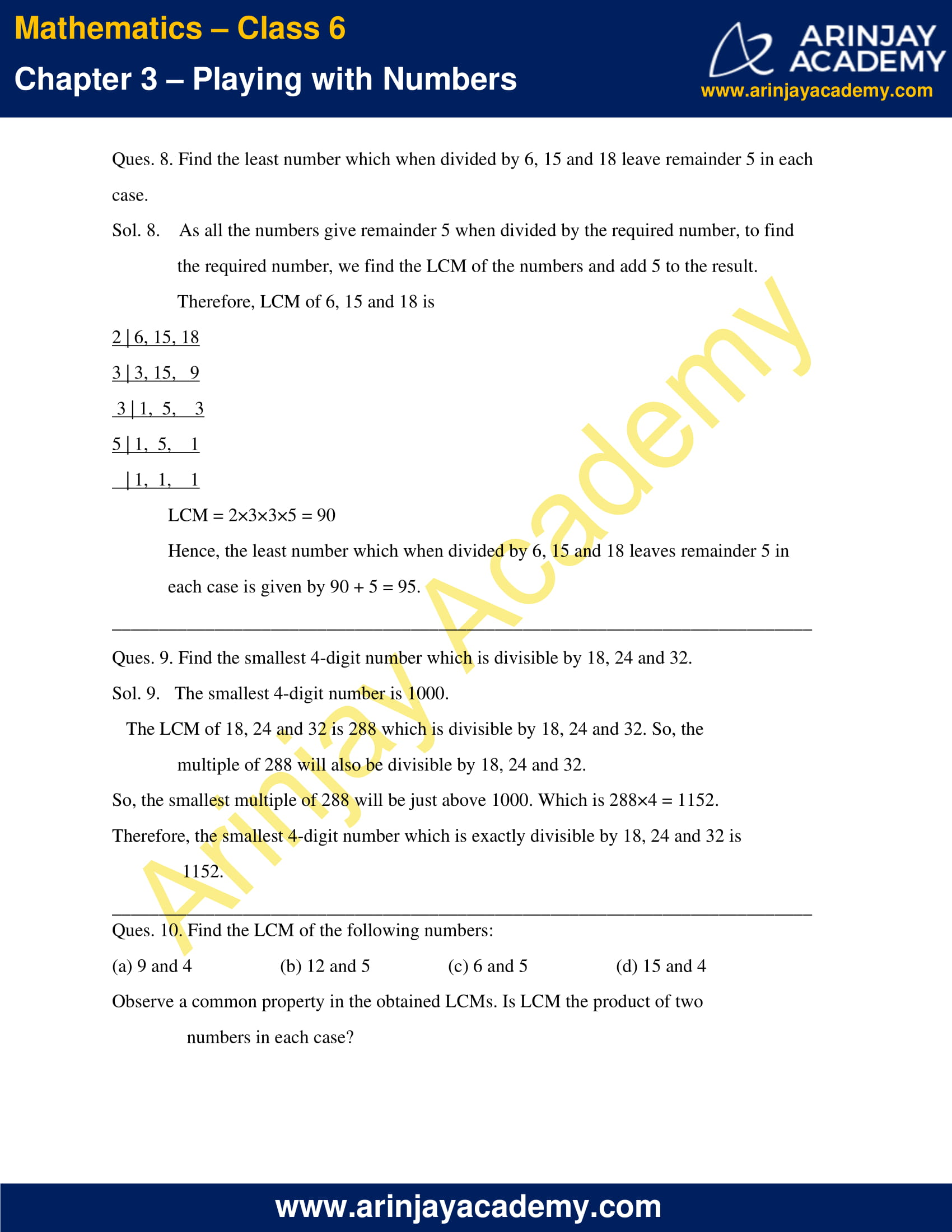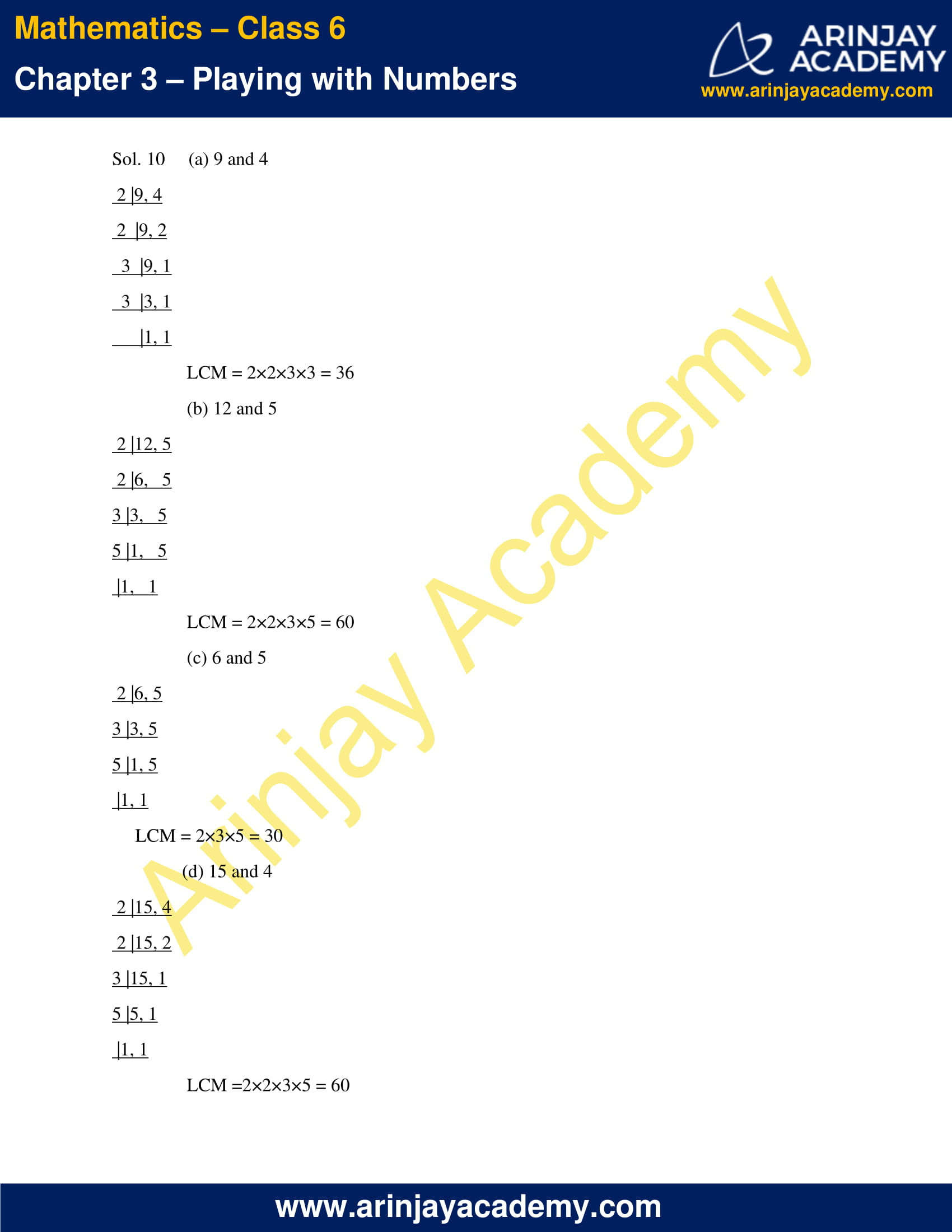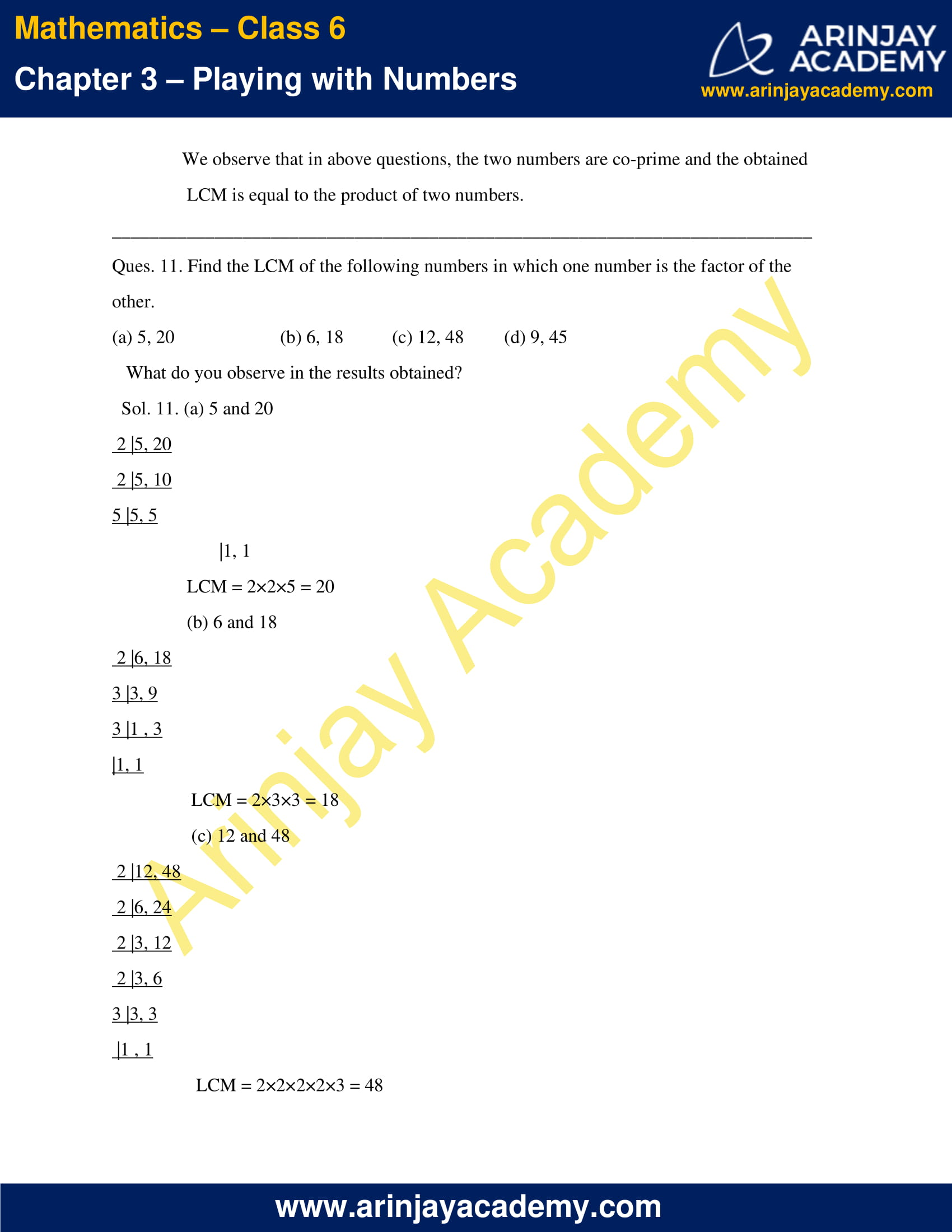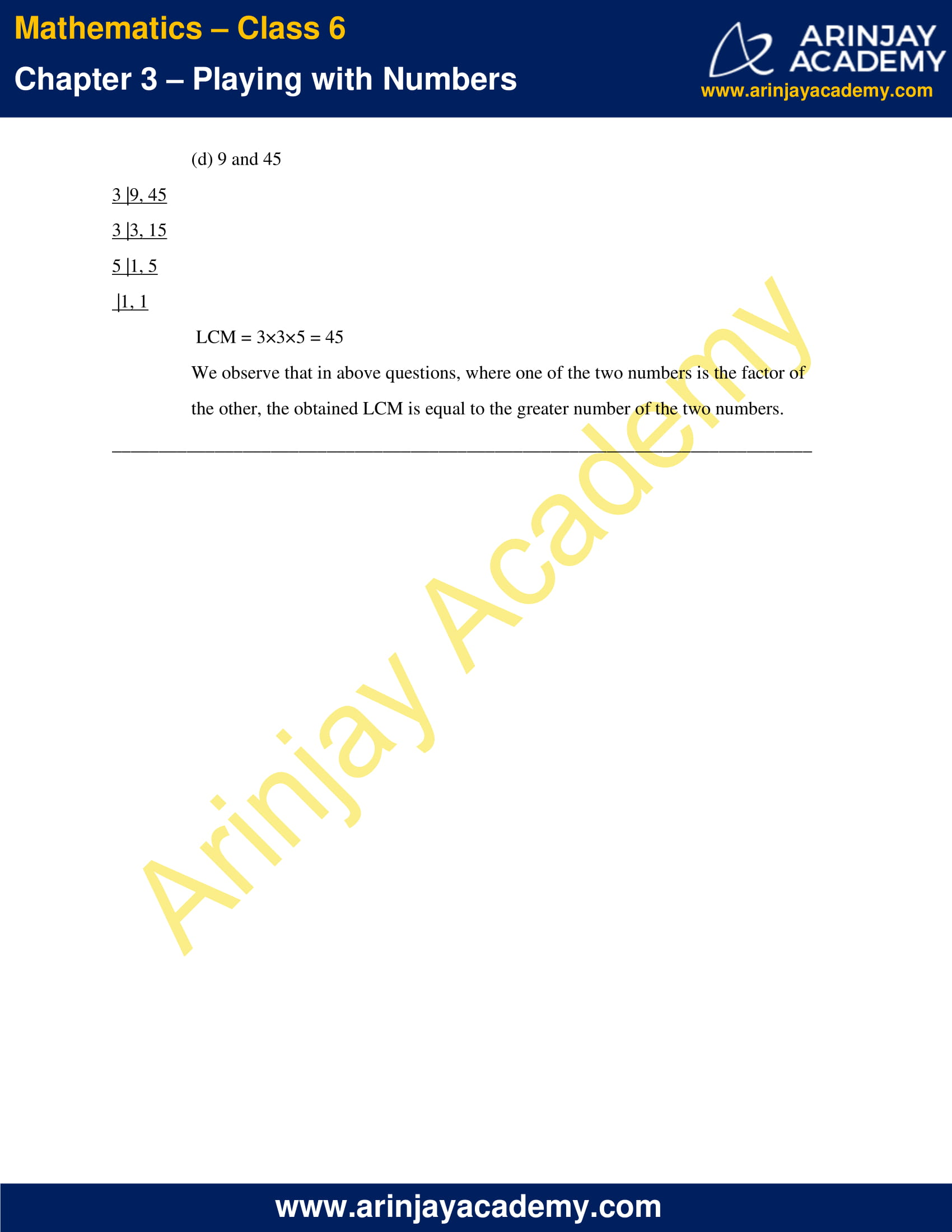### NCERT Solutions for Class 6 Maths Chapter 3 Exercise 3.1

Ques.1. Write all the factors of the following numbers:

(a) 24
(b) 15
(c) 21
(d) 27
(e) 12
(f) 20
(g) 18
(h) 23
(i) 36

Sol.1.    (a) 24 => 1, 2, 3, 4, 6, 8, 12, 24
(b) 15 => 1, 3, 5, 15
(c) 21 => 1, 3, 7, 21
(d) 27 => 1, 3, 9, 27
(e) 12 => 1, 2, 3, 4, 6, 12
(f)  20 => 1, 2, 4, 5, 10, 20
(g) 18 => 1, 2, 3, 6, 9, 18
(h) 23 => 1, 23
(i)  36 => 1, 2, 3, 4, 6, 9, 12, 18, 36

Ques.2. Write first five multiples of:

(a) 5
(b) 8
(c) 9

Sol.2.    (a) 5 × 1 = 5
5 × 2 = 10
5 × 3 = 15
5 × 4 = 20
5 × 5 = 25
Hence, first five multiples of 5 are 5, 10, 15, 20, 25.

(b) 8 × 1 = 8
8 × 2 = 16
8 × 3 = 24
8 × 4 = 32
8 × 5 = 40
Hence, first five multiples of 8 are 8, 16, 24, 32, 40.

(c) 9 × 1 = 9
9 × 2 = 18
9 × 3 = 27
9 × 4 = 36
9 × 5 = 45
Hence, first five multiples of 9 are 9, 18, 27, 36, 45.

Ques.3. Match the items in column 1 with the items in column 2.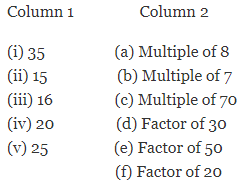Sol.3.   (i) 35 ⇒  (b) Multiple of 7
(7 × 5 = 35)

(ii) 15 ⇒ (d) Factor of 30
(30 ÷ 2 = 15)

(iii) 16 ⇒ (a) Multiple of 8
(8× 2 = 16)

(iv) 20 ⇒ (f) Factor of 20
(20 ÷ 1 = 20)

(v) 25 ⇒ (e) Factor of 50
(50 ÷ 2 = 25)

Ques.4. Find all the multiples of 9 upto 100.

Sol.4.    9 × 1 = 9
9 × 2 = 18
9 × 3 = 27
9 × 4 = 36
9 × 5 = 45
9 × 6 = 54
9 × 7 = 63
9 × 8 = 72
9 × 9 = 81
9 ×10 = 90
9 ×11 = 99
Hence, all the multiples of 9 upto 100 are, 9, 18, 27, 36, 45, 54, 63, 72, 81, 90, 99.

### Class 6 Maths NCERT Solutions Chapter 3 – Exercise 3.2

Ques.1. What is the sum of any two (a) Odd numbers? (b) Even numbers?
Sol.1.    (a) The sum of any two odd numbers is always even.
(b) The sum of any two even numbers is always even.

Ques.2. State whether the following statements are True or False:

(a) The sum of three odd numbers is even.
(b) The sum of two odd numbers and one even number is even.
(c) The product of three odd numbers is odd.
(d) If an even number is divided by 2, the quotient is always odd.
(e) All prime numbers are odd.
(f) Prime numbers do not have any factors.
(g) Sum of two prime numbers is always even.
(h) 2 is the only even prime number.
(i) All even numbers are composite numbers.
(j) The product of two even numbers is always even.

Sol.2.    (a) False ⇒ The sum of two odd numbers gives an even number. Further, the sum of the even number and the third odd number is an odd number. Hence, the sum of three odd numbers is odd.

(b) True ⇒ The sum of two odd numbers is even. Further the sum of this even number with one even number is also even.

(c) True ⇒ The product of two odd numbers is odd. Further the product of this odd number with another odd number is also odd.

(d) False ⇒ Any even number when divided by 2, gives an even or odd quotient not always an odd quotient.

(e) False ⇒ 2 is an even prime number.

(f) False ⇒ Prime numbers have two factors, 1 and the number itself.

(g) False ⇒ Since, 2 is a prime number. Its addition to any other odd prime number gives an odd number.

(h) True ⇒ 2 is the only even prime number.

(i) False ⇒ 2 is an even prime number.

(j) True ⇒ The product of two even numbers is always even.

Ques.3. The numbers 13 and 31 are prime numbers. Both these numbers have same digits 1 and 3. Find such pairs of prime numbers upto 100.

Sol.3. 17 and 71,
37 and 73,
79 and 97

Ques.4. Write down separately the prime and composite numbers less than 20.

Sol.4.     Prime numbers less than 20 are:
2, 3, 5, 7, 11, 13, 17, 19.
Composite numbers less than 20 are:
4, 6, 8, 9, 10, 12, 14, 15, 16, 18.

Ques.5. What is the greatest prime number between 1 and 10?

Sol.5.     Prime numbers between 1 and 10 are 2, 3, 5, 7.
Out of these, the greatest prime number is 7.

Ques.6. Express the following as the sum of two odd primes.

(a) 44
(b) 36
(c) 24
(d) 18

Sol.6. (a) 44 = 3 + 41
= 7 + 37

(b) 36 = 5 + 31
= 7 + 29
= 13 + 23
= 17 + 19

(c) 24 = 5 + 19
= 7 + 17
= 11 + 13

(d) 18 = 5 + 13
= 7 + 11

Ques.7. Give three pairs of prime numbers whose difference is 2.
[Remark : Two prime numbers whose difference is 2 are called twin primes].

Sol.7.    The three pairs of prime numbers whose difference is 2 are:
3 and 5, 5 and 7, 11 and 13.

Ques.8. Which of the following numbers are prime?

(a) 23
(b) 51
(c) 37
(d) 26

Sol.8. (a) 23 is a prime number.
(b) 51 is a composite number with factors 1, 3, 17, 51.
(c) 37 is a prime number.
(d) 26 is a composite number with factors 1, 2, 13, 26.

Ques.9. Write seven consecutive composite numbers less than 100 so that there is no prime number between them.

Sol.9.    The seven consecutive composite numbers less than 100 so that there is no prime number between them, are as follows:
90, 91, 92, 93, 94, 95, 96.

Ques.10. Express each of the following numbers as the sum of three odd primes:

(a) 21
(b) 31
(c) 53
(d) 61

Sol.10. (a) 21 = 3 + 5 + 13
= 3 + 7 + 11

(b) 31 = 3 + 11 + 17
= 3 + 5 + 23
= 5 + 7 + 19
= 7 + 11 + 13

(c) 53 = 13 + 17 + 23

(d) 61 = 7 + 13 + 41

Ques.11. Write five pairs of prime numbers less than 20 whose sum is divisible by 5.
(Hint: 3+7 = 10)

Sol.11. Five pairs of prime numbers less than 20 whose sum is divisible by 5 are:
2 and 3, 3 and 7, 13 and 2, 7 and 13, 3 and 17.

Ques.12. Fill in the blanks :

(a) A number which has only two factors is called a ______.
(b) A number which has more than two factors is called a ______.
(c) 1 is neither ______ nor ______.
(d) The smallest prime number is ______.
(e) The smallest composite number is _____.
(f) The smallest even number is ______.

Sol.12. (a) A number which has only two factors is called a prime number.
(b) A number which has more than two factors is called a composite number.
(c) 1 is neither prime number nor composite number.
(d) The smallest prime number is 2.
(e) The smallest composite number is 4.
(f) The smallest even number is 2.

### NCERT Solutions for Class 6 Maths Chapter 3 Exercise 3.3

Ques.1. Using divisibility tests, determine which of the following numbers are divisible by 2; by 3; by 4; by 5; by 6; by 8; by 9; by 10; by 11 (say, yes or no):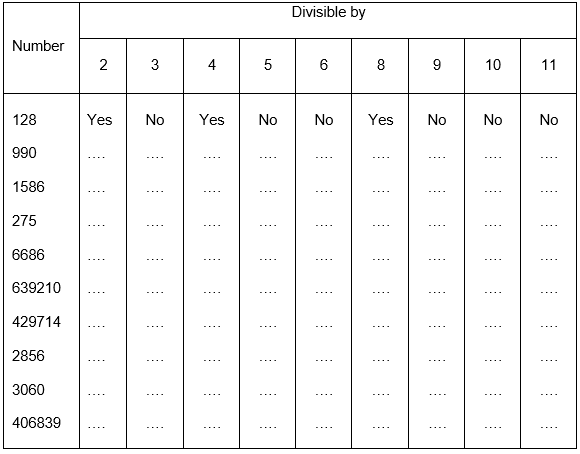Sol.1.    Divisibility by 2: A number is divisible by 2 if it has any of the digits 0, 2, 4, 6 or 8 in its ones place.

Divisibility by 3: If the sum of the digits is a multiple of 3, then the number is divisible by 3.

Divisibility by 4: A number with 3 or more digits is divisible by 4 if the number formed by its last two digits (i.e. ones and tens) is divisible by 4.

Divisibility by 5: A number is divisible by 5 if it has 5 or 0 at its ones place.

Divisibility by 6: If a number is divisible by 2 and 3 both then it is divisible by 6 also.

Divisibility by 8: A number with 4 or more digits is divisible by 8, if the number formed by the last three digits is divisible by 8.

Divisibility by 9: If the sum of the digits of a number is divisible by 9, then the number itself is divisible by 9.

Divisibility by 10: A number is divisible by 10 if it has 0 at its ones place.

Divisibility by 11: If the difference between the sum of the digits at odd places (from the right) and the sum of the digits at even places (from the right) of the number is either 0 or divisible by 11, then the number is divisible by 11.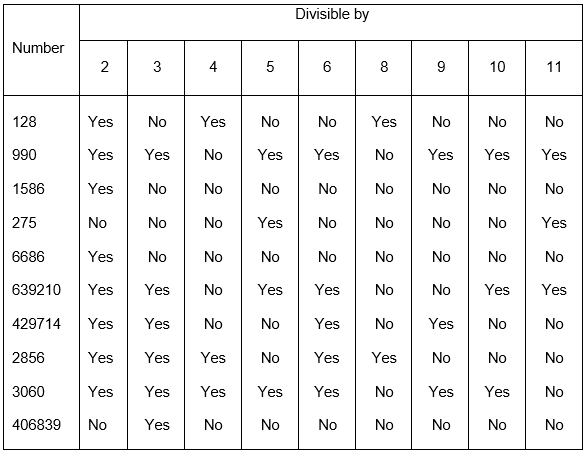Ques. 2. Using divisibility tests, determine which of the following numbers are divisible by 4; by 8:

(a) 572
(b) 726352
(c) 5500
(d) 6000
(e) 12159
(f) 14560
(g) 21084
(h) 31795072
(i) 1700
(j) 2150

Sol.2. (a) Divisibility by 4: The last 2 digits of 572 are 72 which is divisible by 4, hence, 572 is divisible by 4.
Divisibility by 8: The last 3 digits of 572 are 572 which is not divisible by 8, hence, 572 is not divisible by 8.

(b) Divisibility by 4: The last 2 digits of 726352 are 52 which is divisible by 4, hence, 726352 is divisible by 4.
Divisibility by 8: The last 3 digits of 726352 are 352 which is divisible by 8, hence, 726352 is divisible by 8.

(c) Divisibility by 4: The last 2 digits of 5500 are 00 which is divisible by 4, hence, 5500 is divisible by 4.
Divisibility by 8: The last 3 digits of 5500 are 500 which is not divisible by 8, hence, 5500 is not divisible by 8.

(d) Divisibility by 4: The last 2 digits of 6000 are 00 which is divisible by 4, hence, 6000 is divisible by 4.
Divisibility by 8: The last 3 digits of 6000 are 000 which is divisible by 8, hence, 6000 is divisible by 8.

(e) Divisibility by 4: The last 2 digits of 12159 are 59 which is not divisible by 4, hence, 12159 is not divisible by 4.
Divisibility by 8: The last 3 digits of 12159 are 159 which is not divisible by 8, hence, 12159 is not divisible by 8.

(f) Divisibility by 4: The last 2 digits of 14560 are 60 which is divisible by 4, hence, 14560 is divisible by 4.
Divisibility by 8: The last 3 digits of 14560 are 560 which is divisible by 8, hence, 14560 is divisible by 8.

(g) Divisibility by 4: The last 2 digits of 21084 are 84 which is divisible by 4, hence, 21084 is divisible by 4.
Divisibility by 8: The last 3 digits of 21084 are 084 which is not divisible by 8, hence, 21084 is not divisible by 8.

(h) Divisibility by 4: The last 2 digits of 31795072 are 72 which is divisible by 4, hence, 31795072 is divisible by 4.
Divisibility by 8: The last 3 digits of 31795072 are 072 which is divisible by 8, hence, 31795072 is divisible by 8.

(i) Divisibility by 4: The last 2 digits of 1700 are 00 which is divisible by 4, hence, 1700 is divisible by 4.
Divisibility by 8: The last 3 digits of 1700 are 700 which is not divisible by 8, hence, 1700 is not divisible by 8.

(j) Divisibility by 4: The last 2 digits of 2150 are 50 which is not divisible by 4, hence, 2150 is not divisible by 4.
Divisibility by 8: The last 3 digits of 2150 are 150 which is not divisible by 8, hence, 2150 is not divisible by 8.

Ques.3. Using divisibility tests, determine which of following numbers are divisible by 6:

(a) 297144
(b) 1258
(c) 4335
(d) 61233
(e) 901352
(f) 438750
(g) 1790184
(h) 12583
(i) 639210
(j) 17852

Sol.3.   (a) 297144 is divisible by 2 as the last digit is 4.
The sum of digits of 297144 is 2+9+7+1+4+4=27 which is divisible by 3, hence, 297144 is divisible by 3 as well.
Hence, 297144 is divisible by 2 and 3. Therefore, it is also divisible by 6.

(b) 1258 is divisible by 2 as the last digit is 8.
The sum of digits of 1258 is 1+2+5+8=16 which is not divisible by 3, hence, 1258 is not divisible by 3.
Hence, 1258 is divisible by 2 but not divisible by 3. Therefore, 1258 is not divisible by 6.

(c) 4335 is not divisible by 2 as the last digit is 5.
The sum of digits of 4335 is 4+3+3+5=15 which is divisible by 3, hence, 4335 is also divisible by 3
Hence, 4335 is divisible by 3 but not by 2. Therefore, 4335 is not divisible by 6.

(d) 61233 is not divisible by 2 as the last digit is 3.
The sum of digits of 61233 is 6+1+2+3+3=15 which is divisible by 3, hence, 61233 is divisible by 3.
Hence, 61233 is divisible by 3 but not by 2. Therefore, 61233 is not divisible by 6.

(e) 901352 is divisible by 2 as the last digit is 2.
The sum of digits of 901352 is 1+2+5+8=16 which is not divisible by 3, hence, 1258 is not divisible by 3.
Hence, 1258 is divisible by 2 but not by 3. Therefore, 1258 is not divisible by 6.

(f) 438750 is divisible by 2 as the last digit is 0.
The sum of digits of 438750 is 4+3+8+7+5+0=27 which is divisible by 3, hence, 438750 is divisible by 3.
Hence, 438750 is divisible by 2 and 3. Therefore, 438750 is also divisible by 6.

(g) 1790184 is divisible by 2 as the last digit is 4.
The sum of digits of 1790184 is 1+7+9+0+1+8+4=30 which is divisible by 3, hence, 1790184 is divisible by 3.
Hence, 1790184 is divisible by 2 and 3. Therefore, 1790184 is also divisible by 6.

(h)  12583 is not divisible by 2 as the last digit is 3.
The sum of digits of 12583 is 1+2+5+8+3= 19 which is not divisible by 3, hence, 12583 is not divisible by 3.
Hence, 12583 is not divisible by 2 and 3. Therefore, 12853 is not divisible by 6.

(i)   639210 is divisible by 2 as the last digit is 0.
The sum of digits of 639210 is 6+3+9+2+1+0=21 which is divisible by 3, hence, 639210 is divisible by 3.
Hence 639210 is divisible by 2 and 3. Therefore, 639210 is also divisible by 6.

(j)  17852 is divisible by 2 as the last digit is 2.
The sum of digits of 17852 is 1+7+8+5+2=23 which is not divisible by 3, hence, 17852 is not divisible by 3.
Hence 17852 is divisible by 2 but not divisible by 3. Therefore, 17852 is not divisible by 6.

Ques.4. Using divisibility tests, determine which of the following numbers are divisible by 11:

(a) 5445
(b) 10824
(c) 7138965
(d) 70169308
(e) 10000001
(f) 901153

Sol.4. (a) The sum of digits at odd places from the right   = 5+4 = 9
The sum of digits at even places from the right = 4+5 = 9
Difference = 9 –  9 = 0
Hence, 5445 is divisible by 11.

(b) The sum of digits at odd places from the right   = 4+8+1 = 13
The sum of digits at even places from the right = 2+0 = 2
Difference = 13 –  2 = 11
Hence, 10824 is divisible by 11.

(c) The sum of digits at odd places from the right   = 5+9+3+7 = 24
The sum of digits at even places from the right = 6+8+1 = 15
Difference = 24 – 15 = 9
Hence, 7138965 is not divisible by 11.

(d) The sum of digits at odd places from the right   = 8+3+6+0 = 17
The sum of digits at even places from the right = 0+9+1+7 = 17
Difference = 17 – 17 = 0
Hence, 70169308 is divisible by 11.

(e) The sum of digits at odd places from the right   = 1+0+0+0 = 1
The sum of digits at even places from the right = 0+0+0+1 = 1
Difference = 1 – 1 = 0
Hence, 10000001 is divisible by 11.

(f)  The sum of digits at odd places from the right   = 3+1+0 = 4
The sum of digits at even places from the right = 5+1+9 = 15
Difference = 15 – 4 = 11
Hence, 901153 is divisible by 11.

Ques.5. Write the smallest digit and the greatest digit in the blank space of each of the following numbers so that the number formed is divisible by 3 :

(a) __ 6724              (b) 4765 __ 2

Sol.5.      (a) The sum of existing digits = 6+7+2+4 = 19
The smallest digit to be added to this sum to make it divisible by 3 = 2
19+2=21 which is divisible by 3. Hence, 26724 is divisible by 3.
The greatest digit to be added to this sum to make it divisible by 3 = 8
19+8=27 which is divisible by 3. Hence, 86724 is divisible by 3.

(b) The sum of existing digits = 4+7+6+5+2 = 24
The smallest digit to be added to this sum to make it divisible by 3 = 0
24+0=24 which is divisible by 3. Hence, 476502 is divisible by 3.
The greatest digit to be added to this sum to make it divisible by 3 = 9
24+9=33 which is divisible by 3. Hence, 476592 is divisible by 3.

Ques.6. Write a digit in the blank space of each of the following numbers so that the number formed is divisible by 11:

(a) 92 __ 389
(b) 8 __ 9484

Sol.6.    (a) The sum of digits at odd places from the right   = 9+3+2 = 14
The sum of digits at even places from the right = 8+9 = 17
Difference = 17 – 14 = 3
To make it divisible by 11, 8 should be added at the even place, such that
17+8=25.
Now, the difference between the sum of digits at odd and even places= 25-14 = 11
Hence, 928389 is divisible by 11.

(b) The sum of digits at odd places from the right   = 4+4 = 8
The sum of digits at even places from the right = 8+9+8 = 25
Difference = 25 – 8 = 17
To make it divisible by 11, 6 should be added at the odd place, such that
8+6=14.
Now, the difference between the sum of digits at odd and even places= 25-14 = 11
Hence, 869484 is divisible by 11.

### NCERT Solutions for Class 6 Maths Chapter 3 Exercise 3.4

Ques.1. Find the common factors of:

(a) 20 and 28
(b) 15 and 25
(c) 35 and 50
(d) 56 and 120

Sol.1.    (a) Factors of 20 are 1, 2, 4, 5, 10, 20.
Factors of 28 are 1, 2, 4, 7, 14, 28.
Common factors of 20 and 28 are 1, 2 and 4.

(b) Factors of 15 are 1, 3, 5, 15.
Factors of 25 are 1, 5, 25.
Common factors of 15 and 25 are 1 and 5.

(c) Factors of 35 are 1, 5, 7, 35.
Factors of 50 are 1, 2, 5, 10, 25, 50.
Common factors of 35 and 50 are 1 and 5.

(d) Factors of 56 are 1, 2, 4, 7, 8, 14, 28, 56.
Factors of 120 are 1, 2, 3, 4, 5, 6, 8, 10, 12, 15, 20, 24, 30, 40, 60, 120.
Common factors of 56 and 120 are 1, 2, 4 and 8.

Ques.2. Find the common factors of:

(a) 4, 8 and 12
(b) 5, 15 and 25

Sol.2.    (a) Factors of 4 are 1, 2, 4.
Factors of 8 are 1, 2, 4, 8.
Factors of 12 are 1, 2, 3, 4, 6, 12.
Common factors of 4, 8 and 12 are 1, 2 and 4.

(b) Factors of 5 are 1, 5.
Factors of 15 are 1, 3, 5, 15.
Factors of 25 are 1, 5, 25.
Common factors of 5, 15 and 25 are 1 and 5.

Ques.3. Find first three common multiples of:

(a) 6 and 8
(b) 12 and 18

Sol.3.    (a) Multiples of 6 = 6, 12, 18, 24, 30, 36, 42, 48, 54, 60, 66, 72…
Multiples of 8 = 8, 16, 24, 32, 40, 48, 56, 64, 72, 80, 88….
First three common multiples of 6 and 8 = 24, 48, 72.

(b)  Multiples of 12 = 12, 24, 36, 48, 60, 72, 84, 96, 108…
Multiples of 18 = 18, 36, 54, 72, 90, 108…
First three common multiples of 12 and 18 = 36, 72, 108.

Ques.4. Write all the numbers less than 100 which are common multiples of 3 and 4.

Sol.4.     Multiples of 3 = 3, 6, 9, 12, 15, 18, 21, 24, 27, 30, 33, 36, 39, 42, 45, 48, 51, 54, 57, 60, 63, 66, 69, 72, 75, 78, 81, 84, 87, 90, 93, 96, 99…
Multiples of 4 = 4, 8, 12, 16, 20, 24, 28, 32, 36, 40, 44, 48, 52, 56, 60, 64, 68, 72,76, 80, 84, 88, 92, 96, 100.
Common multiples of 3 and 4 (less than 100) = 12, 24, 36, 48, 60, 72, 84, 96.

Ques.5. Which of the following numbers are co-prime?

(a) 18 and 35
(b) 15 and 37
(c) 30 and 415
(d) 17 and 68
(e) 216 and 215
(f) 81 and 16

Sol.5.    (a) Factors of 18 are 1, 2, 3, 6, 9, 18.
Factors of 35 are 1, 5, 7, 35.
Common factors of 18 and 35 is 1.
Hence, 18 and 35 are co-prime.

(b) Factors of 15 are 1, 3, 5, 15.
Factors of 37 are 1, 37.
Common factors of 15 and 37 is 1.
Hence, 15 and 37 are co-prime.

(c) Factors of 30 are 1, 2, 3, 5, 6, 10, 15, 30.
Factors of 415 are 1, 5, 83, 415.
Common factors of 30 and 415 are 1 and 5.
Hence, 30 and 415 are not co-prime.

(d) Factors of 17 are 1, 17.
Factors of 68 are 1, 2, 4, 17, 34, 68.
Common factors of 17 and 68 are 1 and 17.
Hence, 17 and 68 are not co-prime.

(e) Factors of 216 are 1, 2, 3, 4, 6, 8, 9, 12, 18, 24, 27, 36, 54, 72, 108, 216.
Factors of 215 are 1, 5, 43, 215.
Common factors of 216 and 215 is 1.
Hence, 216 and 215 are co-prime.

(f)   Factors of 81 are 1, 3, 9, 27, 81.
Factors of 16 are 1, 2, 4, 8, 16
Common factors of 81 and 16 is just 1.
Hence, 81 and 16 are co-prime.

Ques.6. A number is divisible by both 5 and 12. By which other number will that number be always divisible?

Sol.6.    If a number is divisible by 5 and 12 both, then it will always be divisible by the least common multiple of 5 and 12, i.e., 60.

Ques.7. A number is divisible by 12. By what other numbers will that number be divisible?

Sol.7.    If a number is divisible by 12, then it will be divisible by all the factors of 12, i.e., 1, 2, 3, 4 and 6.

### NCERT Solutions for Class 6 Maths Chapter 3 Exercise 3.5

Ques.1. Which of the following statements are true?

(a) If a number is divisible by 3, it must be divisible by 9.
(b) If a number is divisible by 9, it must be divisible by 3.
(c) A number is divisible by 18, if it is divisible by both 3 and 6.
(d) If a number is divisible by 9 and 10 both, then it must be divisible by 90.
(e) If two numbers are co-primes, at least one of them must be prime.
(f) All numbers which are divisible by 4 must also be divisible by 8.
(g) All numbers which are divisible by 8 must also be divisible by 4.
(h) If a number exactly divides two numbers separately, it must exactly divide their sum.
(i) If a number exactly divides the sum of two numbers, it must exactly divide the two numbers separately.

Sol.1. (a) False (6 is divisible by 3, but not by 9)
(b) True (3 is a factor of 9. Hence, any number divisible by 9 is also divisible by 3.)
(c) False (12 is divisible by 3 and 6 both, but not by 18.)
(d) True (If a number is divisible by 9 and 10 both, then it must be divisible by their LCM, i.e., 90.)
(e) False (15 and 16 are co-prime and neither of them is prime.)
(f)  False (12 is divisible by 4 but not by 8.)
(g) True (4 is a factor of 8. Hence, any number divisible by 8 is also divisible by 4.)
(h) True (By distributive property of multiplication over addition, If a number exactly divides two numbers separately, it can be taken as the common factor, and hence, divide their sum exactly as well.)
(i)  False (This is not necessary. For example, 6 exactly divides the sum of 8 and 4, i.e., 12. But, 6 neither divides 8 nor 4, separately.)

Ques.2. Here are two different factor trees for 60. Write the missing numbers.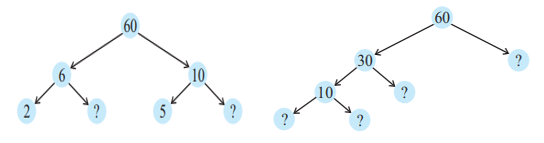Sol.2.   60 = 6 × 10
6 = 2 × 3
10 = 5 × 2

In second tree diagram,
60 = 30 × 2
30 = 10 × 3
10 = 2 × 5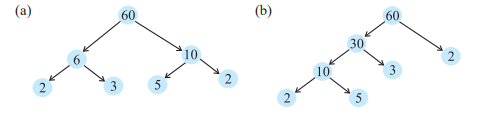Ques.3. Which factors are not included in the prime factorisation of a composite number?

Sol.3.     In the prime factorization of a composite number, 1 and the number itself are not Included.

Ques.4. Write the greatest 4-digit number and express it in terms of its prime factors.

Sol.4.     9999 is the greatest 4-digit number.
In terms of prime factors, 9999 = 3 × 3 × 11 × 101

Ques.5. Write the smallest 5-digit number and express it in the form of its prime factors.

Sol.5.     10000 is the smallest 5-digit number.
In terms of prime factors, 10000 = 2 × 2 × 2 × 2 × 5 × 5 × 5 × 5

Ques.6. Find all the prime factors of 1729 and arrange them in ascending order. Now state the relation, if any; between two consecutive prime factors.

Sol.6.    1729 = 7 × 13 × 19
The relation between two consecutive prime factors is such that they are in arithmetic progression, with the common difference between two consecutive prime factors as 6.

Ques.7. The product of three consecutive numbers is always divisible by 6. Verify this statement with the help of some examples.

Sol.7.     1 × 2 × 3 = 6   …divisible by 6
2 × 3 × 4 = 24  …divisible by 6
3 × 4 × 5 = 60  …divisible by 6
:
:
10 × 11 × 12 = 1320
From above all examples, it is clear that the product of three consecutive numbers is always divisible by 6.

Ques.8. The sum of two consecutive odd numbers is divisible by 4. Verify this statement with the help of some examples.

Sol.8.    1 + 3 = 4     …divisible by 4
3 + 5 = 8       …divisible by 4
5 + 7 = 12      …divisible by 4
7 + 9 = 16      …divisible by 4
:
:
And so on …
From above all examples, it is clear that the sum of two consecutive odd numbers is divisible by 4.

Ques.9. In which of the following expressions, prime factorisation has been done?

(a) 24 = 2 × 3 × 4
(b) 56 = 7 × 2 × 2 × 2
(c) 70 = 2 × 5 × 7
(d) 54 = 2 × 3 × 9

Sol.9. (a) 4 is not a prime factor.
(b) 7 and 2 are prime factors of 56.
(c) 2, 5 and 7 are prime factors of 70.
(d) 9 is not a prime factor.
Hence, prime factorization has been done in option (b) and (c).

Ques.10. Determine if 25110 is divisible by 45.
[Hint : 5 and 9 are co-prime numbers. Test the divisibility of the number by 5 and 9].

Sol.10.  25110 is divisible by 5 as it ends with 0.
It is divisible by 9 as well.
Now, as it is divisible by 5 and 9 both, it must be divisible by their LCM, i.e., 45.
Hence, 25110 is divisible by 45.

Ques.11. 18 is divisible by both 2 and 3. It is also divisible by 2 × 3 = 6. Similarly, a number is divisible by both 4 and 6. Can we say that the number must also be divisible by 4 × 6 = 24? If not, give an example to justify your answer.

Sol.11.  No. As an example, 12 is divisible by both 4 and 6. But, 12 is not divisible by 4 × 6 i.e., 24.

Ques.12. I am the smallest number, having four different prime factors. Can you find me?

Sol.12.    The smallest number having four different prime factors is obtained by finding the product of 4 smallest prime numbers.
Hence, the smallest number is 2 × 3 × 5 ×7 = 210.

### NCERT Solutions for Class 6 Maths Chapter 3 Exercise 3.6

Ques. 1. Find the HCF of the following numbers:

(a) 18, 48
(b) 30, 42
(c) 18, 60
(d) 27, 63
(e) 36, 84
(f) 34, 102
(g) 70, 105, 175
(h) 91, 112, 49
(i) 18, 54, 81
(j) 12, 45, 75

Sol. 1.  (a)18 = 1×2×3×3
48 = 1×2×2×2×2×3
HCF = 2×3 = 6

(b) 30 = 1×2×3×5
42 = 1×2×3×7
HCF = 2×3 = 6

(c) 18 = 1×2×3×3
60 = 1×2×2×3×5
HCF = 2×3 = 6

(d) 27 = 1×3×3×3
63 = 1×3×3×7
HCF = 3×3 = 9

(e) 36 = 1×2×2×3×3
84 = 1×2×2×3×7
HCF = 2×2×3 = 12

(f) 34 = 1×2×17
102 = 1×2×3×17
HCF = 2×17 = 34

(g) 70 = 1×2×5×7
105 = 1×3×5×7
175 = 1×5×5×7
HCF= 5×7 = 35

(h) 91 = 1×7×13
112 = 1×2×2×2×2×7
49 = 1×7×7
HCF = 7

(i) 18 = 1×2×3×3
54 = 1×2×3×3×3
81 = 1×3×3×3×3
HCF = 3×3 = 9

(j) 12 = 1×2×2×3
45 = 1×3×3×5
75 = 1×3×5×5
HCF = 3

Ques. 2. What is the HCF of two consecutive

(a) numbers?
(b) even numbers?
(c) odd numbers?

Sol. 2.(a) Two consecutive numbers do not have any common factor other than 1.
Therefore, HCF of two consecutive numbers is 1.

(b) Two consecutive even numbers have 2 as their common factor.
Therefore, HCF of two consecutive even numbers is 2.

(c) Two consecutive odd numbers do not have any common factor other than 1.
Therefore, HCF of two consecutive odd numbers is 1.

Ques. 3. HCF of co-prime numbers 4 and 15 was found as follows by factorisation:
4 = 2 × 2 and 15 = 3 × 5 since there is no common prime factor, so HCF of 4 and 15 is 0. Is the answer correct? If not, what is the correct HCF?

Sol. 3.    No, this answer is not correct. There is a common factor as 1, even though the two numbers are co-prime. Therefore, the HCF of 4 and 15 is 1.

### NCERT Solutions for Class 6 Maths Chapter 3 Exercise 3.7

Ques. 1. Renu purchases two bags of fertiliser of weights 75 kg and 69 kg. Find the maximum value of weight which can measure the weight of the fertiliser exact number of times.

Sol. 1.   To find the maximum value of weight which can measure the weight of the fertiliser exact number of times we need to find the highest common factor of the weight of both fertilisers.
HCF of 75 and 69
75 = 3×5×5
69 = 3×23
Common factor of 75 and 69 is 3.
HCF = 3
Therefore, 3kg is the maximum value of weight which can measure the weight of the fertiliser exact number of times.

Ques. 2. Three boys step off together from the same spot. Their steps measure 63 cm, 70 cm and 77 cm respectively. What is the minimum distance each should cover so that all can cover the distance in complete steps?

Sol. 2.  To find the minimum distance each should cover so that all can cover the distance in complete steps we need to find the lowest common multiple of their steps.
LCM of 63,70 and 77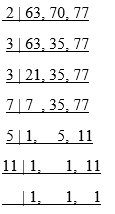L.C.M. = 2×3×3×7×5×11 = 6930 cm
Therefore 6930 cm is the minimum distance each should cover so that all can cover the distance in complete steps.

Ques. 3. The length, breadth and height of a room are 825 cm, 675 cm and 450 cm respectively. Find the longest tape which can measure the three dimensions of the room exactly.

Sol. 3. To find the longest tape which can measure the three dimensions of the room exactly HCF of 825,675 and 450
825 = 3×5×5×11
675 = 3×3×3×5×5
450 = 2×3×3×5×5
Common factor of 825, 675 and 450 is 3×5×5 = 75.
Therefore, the longest tape which can measure the three dimensions of the room exactly is 75cm.

Ques.4. Determine the smallest 3-digit number which is exactly divisible by 6, 8 and 12.

Sol. 4.  The smallest 3-digit number is 100.

The LCM of 6, 8 and 12 is 24 which is divisible by 6,8 and 12. So, the multiple of 24 will also be divisible by 6, 8 and 12.
So, the smallest multiple of 24 will be just above 100. Which is 24×5 = 120.
Therefore, the smallest 3-digit number which is exactly divisible by 6, 8 and 12 is 120.

Ques.5. Determine the greatest 3-digit number exactly divisible by 8, 10 and 12.

Sol. 5.   The greatest 3-digit number is 999.
The LCM of 8, 10 and 12 is 120 which is divisible by 8, 10 and 12. So, the multiple of 120 will also be divisible by 8, 10 and 12.
So, the greatest multiple of 120 will be just below 999. Which is 120×8 = 960.
Therefore, the greatest 3-digit number which is exactly divisible by 8, 10 and 12 is 960.

Ques.6. The traffic lights at three different road crossings change after every 48 seconds, 72 seconds and 108 seconds respectively. If they change simultaneously at 7 a.m., at what time will they change simultaneously again?

Sol. 6.   To find at what time will they change simultaneously again we need to find LCM.
LCM of 48, 72 and 108 is 432.
So, after 432 second or 7 minute 12 second the light will change simultaneously.
Therefore, it will change simultaneously again at 7 minute 12 second passed 7 a.m. or at 07:07:12.

Ques. 7. Three tankers contain 403 litres, 434 litres and 465 litres of diesel respectively. Find the maximum capacity of a container that can measure the diesel of the three containers exact number of times.

Sol. 7.  To Find the maximum capacity of a container that can measure the diesel of the three containers exact number of times we need to find the HCF of litres of diesel in each tanker.
HCF of 403, 434 and 465
403 = 1×13×31
434 = 1×2×7×31
465 = 1×3×5×31
Therefore, HCF of 403, 434 and 465 = 31

Ques. 8. Find the least number which when divided by 6, 15 and 18 leave remainder 5 in each case.

Sol. 8.    As all the numbers give remainder 5 when divided by the required number, to find the required number, we find the LCM of the numbers and add 5 to the result.
Therefore, LCM of 6, 15 and 18 is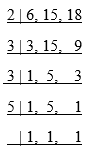LCM = 2×3×3×5 = 90
Hence, the least number which when divided by 6, 15 and 18 leaves remainder 5 in each case is given by 90 + 5 = 95.

Ques. 9. Find the smallest 4-digit number which is divisible by 18, 24 and 32.

Sol. 9.   The smallest 4-digit number is 1000.
The LCM of 18, 24 and 32 is 288 which is divisible by 18, 24 and 32. So, the multiple of 288 will also be divisible by 18, 24 and 32.
So, the smallest multiple of 288 will be just above 1000. Which is 288×4 = 1152.
Therefore, the smallest 4-digit number which is exactly divisible by 18, 24 and 32 is 1152.

Ques. 10. Find the LCM of the following numbers:

(a) 9 and 4
(b) 12 and 5
(c) 6 and 5
(d) 15 and 4

Observe a common property in the obtained LCMs. Is LCM the product of two numbers in each case?

Sol. 10     (a) 9 and 4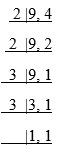LCM = 2×2×3×3 = 36

(b) 12 and 5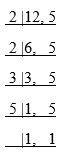LCM = 2×2×3×5 = 60

(c) 6 and 5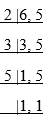LCM = 2×3×5 = 30

(d) 15 and 4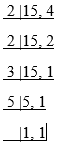LCM =2×2×3×5 = 60

We observe that in above questions, the two numbers are co-prime and the obtained LCM is equal to the product of two numbers.

Ques. 11. Find the LCM of the following numbers in which one number is the factor of the other.

(a) 5, 20
(b) 6, 18
(c) 12, 48
(d) 9, 45

What do you observe in the results obtained?

Sol. 11. (a) 5 and 20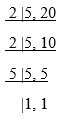LCM = 2×2×5 = 20

(b) 6 and 18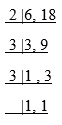LCM = 2×3×3 = 18

(c) 12 and 48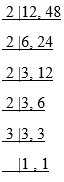LCM = 2×2×2×2×3 = 48

(d) 9 and 45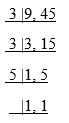LCM = 3×3×5 = 45

We observe that in above questions, where one of the two numbers is the factor of the other, the obtained LCM is equal to the greater number of the two numbers.

With this we come to the end of NCERT Solutions for Class 6 Maths Chapter 3. We hope these helped you study your subject.